Скачать презентацию THE OPERATIONS OF MARKETS DEMAND SUPPLY Dr Michelle

8d21efe5d0d236c852d2582c59ddbdc6.ppt

• Количество слайдов: 94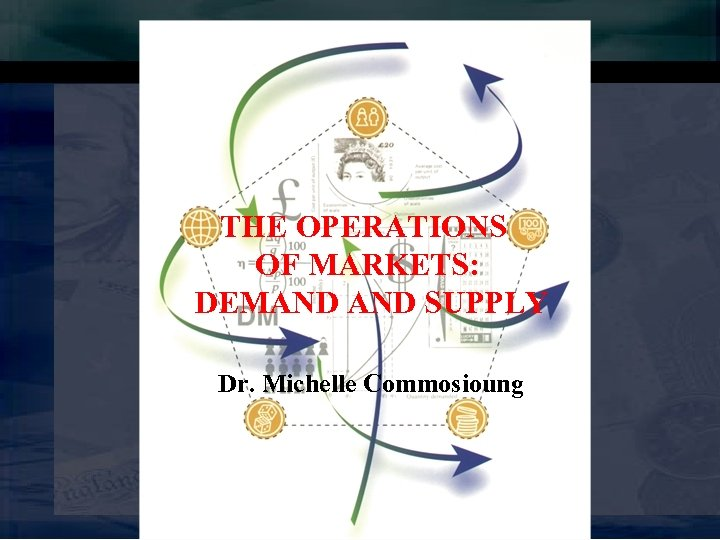THE OPERATIONS OF MARKETS: DEMAND SUPPLY Dr. Michelle Commosioung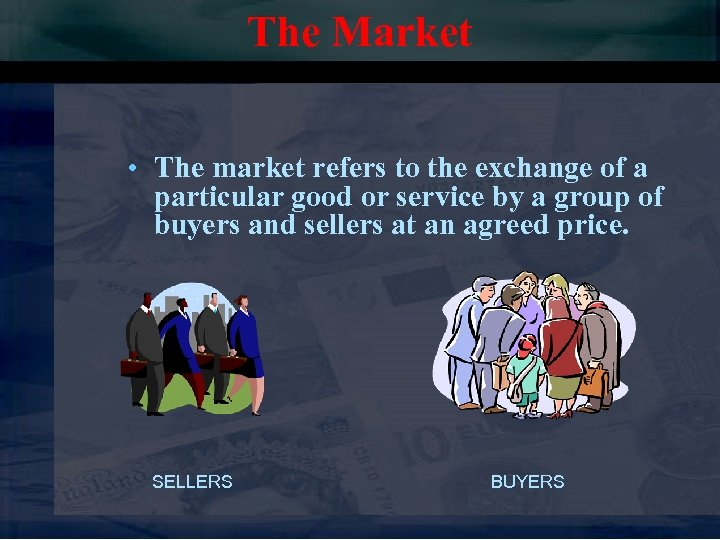The Market • The market refers to the exchange of a particular good or service by a group of buyers and sellers at an agreed price. SELLERS BUYERS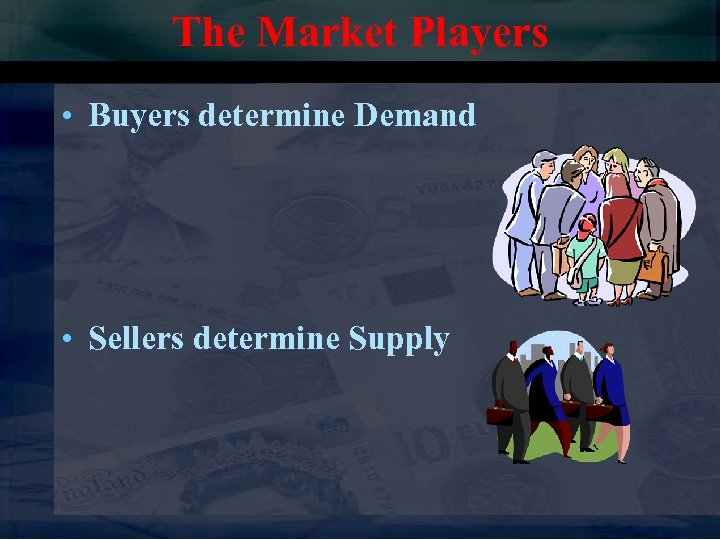The Market Players • Buyers determine Demand • Sellers determine Supply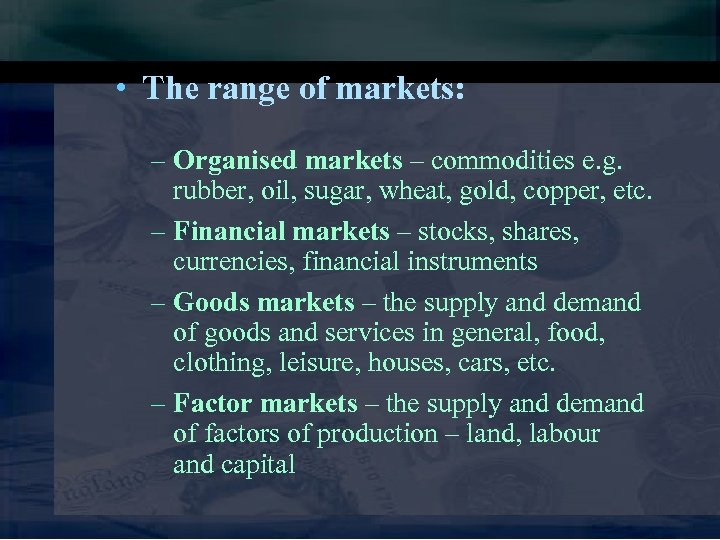• The range of markets: – Organised markets – commodities e. g. rubber, oil, sugar, wheat, gold, copper, etc. – Financial markets – stocks, shares, currencies, financial instruments – Goods markets – the supply and demand of goods and services in general, food, clothing, leisure, houses, cars, etc. – Factor markets – the supply and demand of factors of production – land, labour and capital• A market does NOT have to be a physical place like a shop • The market place consists of all those who have items/services for sale and all those who are interested in buying those items/services • Many businesses have global markets because of the developments in technology – e. g. www. amazon. com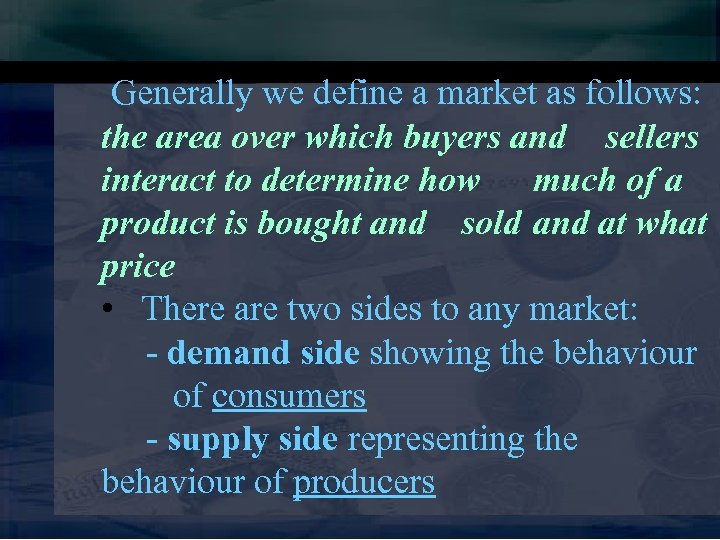Generally we define a market as follows: the area over which buyers and sellers interact to determine how much of a product is bought and sold and at what price • There are two sides to any market: - demand side showing the behaviour of consumers - supply side representing the behaviour of producers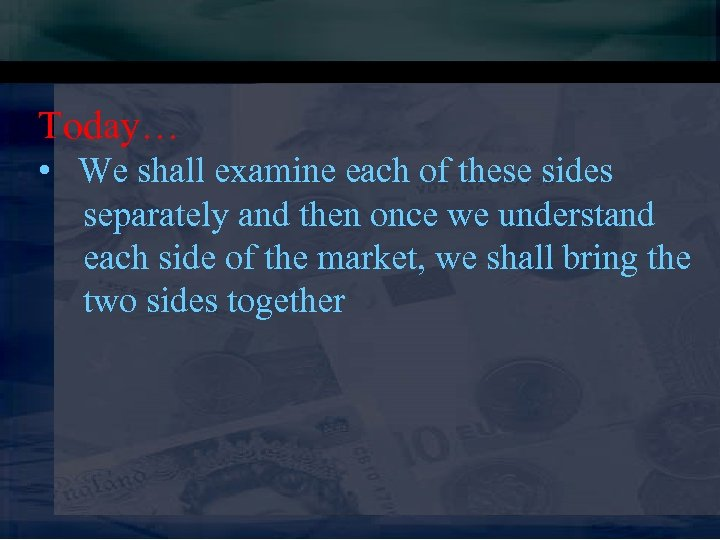Today… • We shall examine each of these sides separately and then once we understand each side of the market, we shall bring the two sides together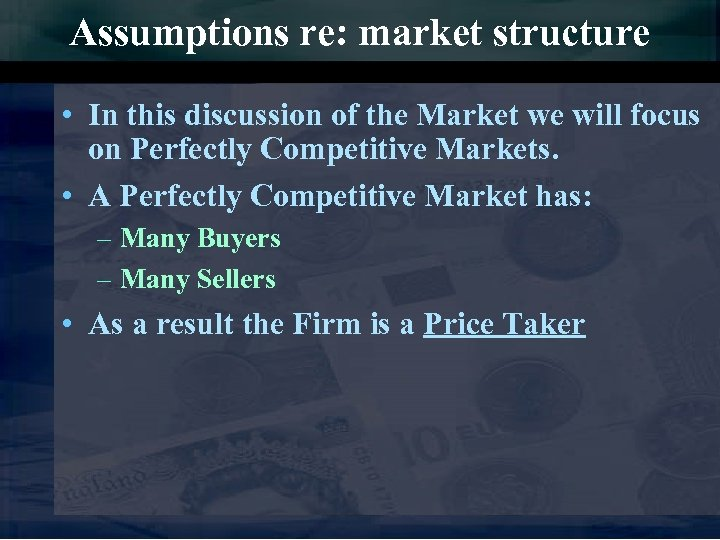Assumptions re: market structure • In this discussion of the Market we will focus on Perfectly Competitive Markets. • A Perfectly Competitive Market has: – Many Buyers – Many Sellers • As a result the Firm is a Price Taker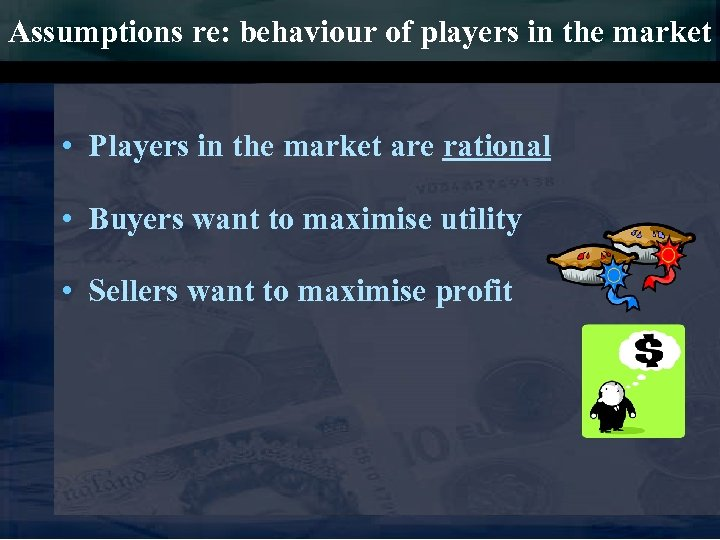Assumptions re: behaviour of players in the market • Players in the market are rational • Buyers want to maximise utility • Sellers want to maximise profit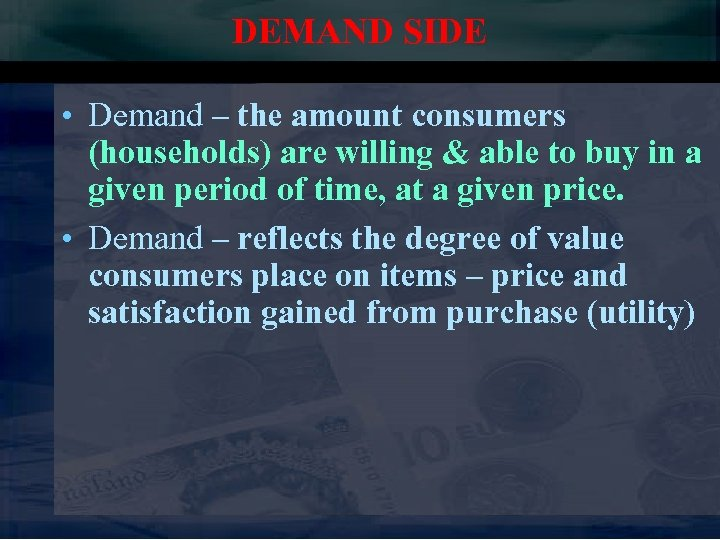DEMAND SIDE • Demand – the amount consumers (households) are willing & able to buy in a given period of time, at a given price. • Demand – reflects the degree of value consumers place on items – price and satisfaction gained from purchase (utility)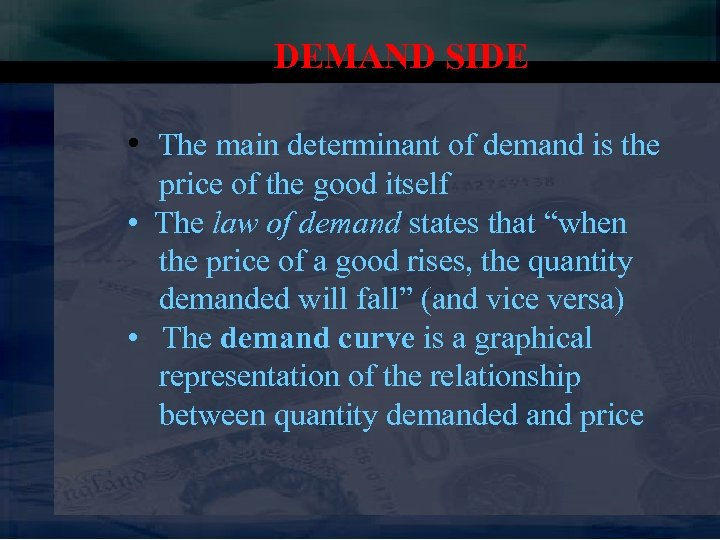DEMAND SIDE • The main determinant of demand is the price of the good itself • The law of demand states that “when the price of a good rises, the quantity demanded will fall” (and vice versa) • The demand curve is a graphical representation of the relationship between quantity demanded and price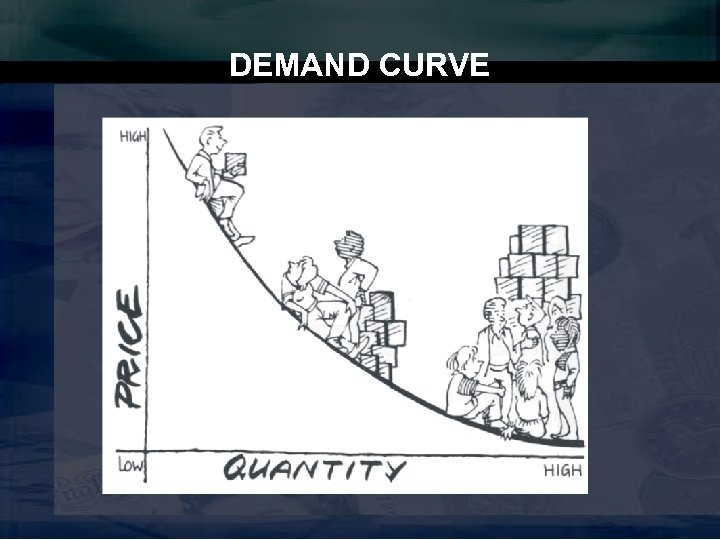DEMAND CURVE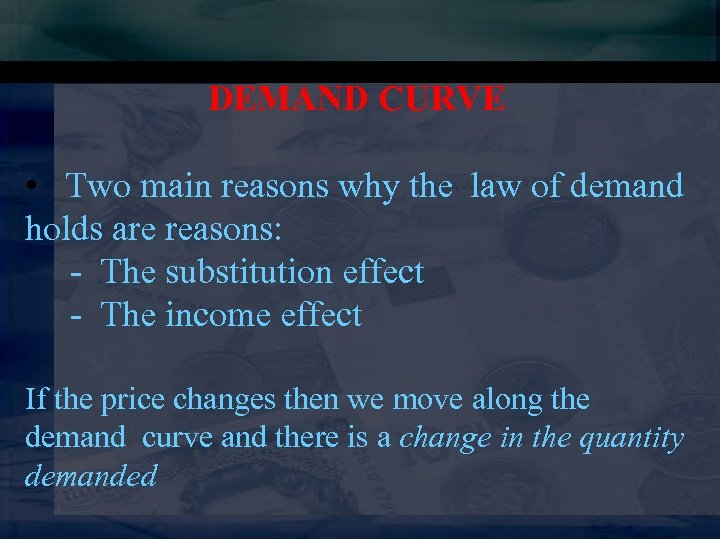DEMAND CURVE • Two main reasons why the law of demand holds are reasons: - The substitution effect - The income effect If the price changes then we move along the demand curve and there is a change in the quantity demanded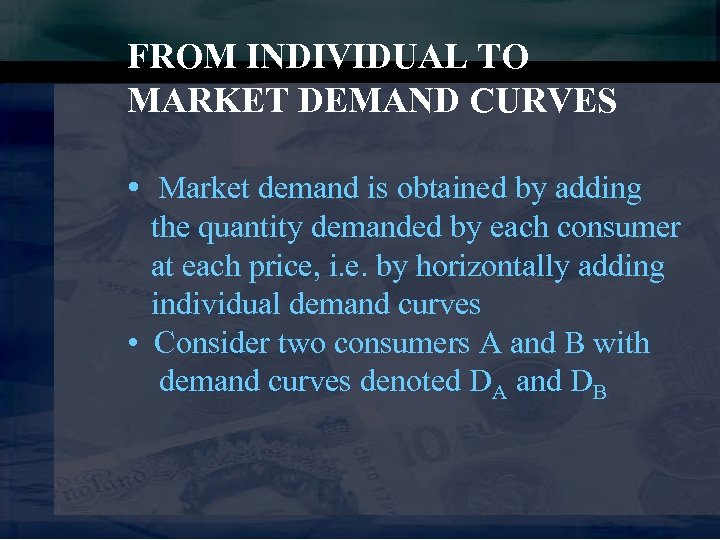FROM INDIVIDUAL TO MARKET DEMAND CURVES • Market demand is obtained by adding the quantity demanded by each consumer at each price, i. e. by horizontally adding individual demand curves • Consider two consumers A and B with demand curves denoted DA and DB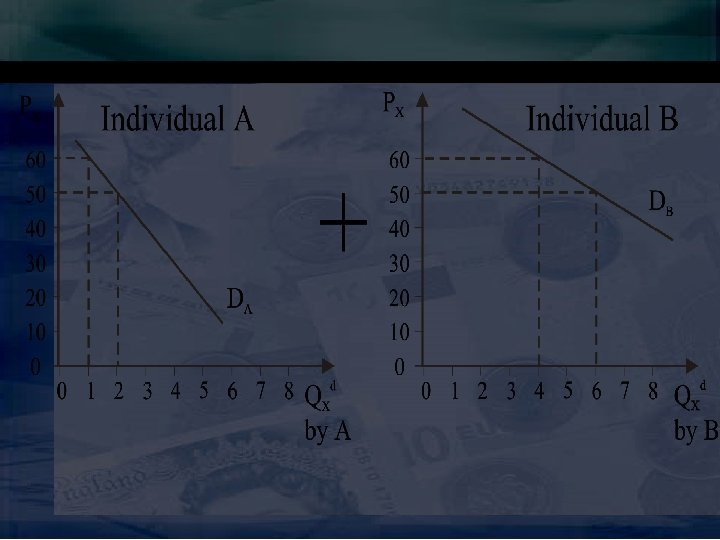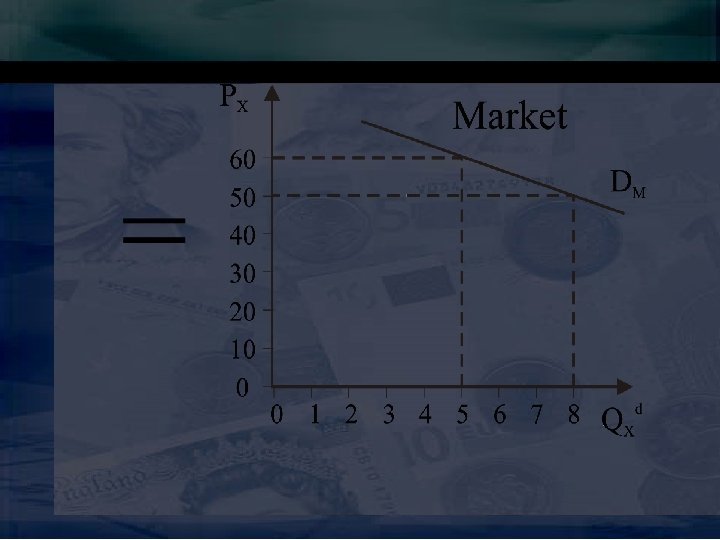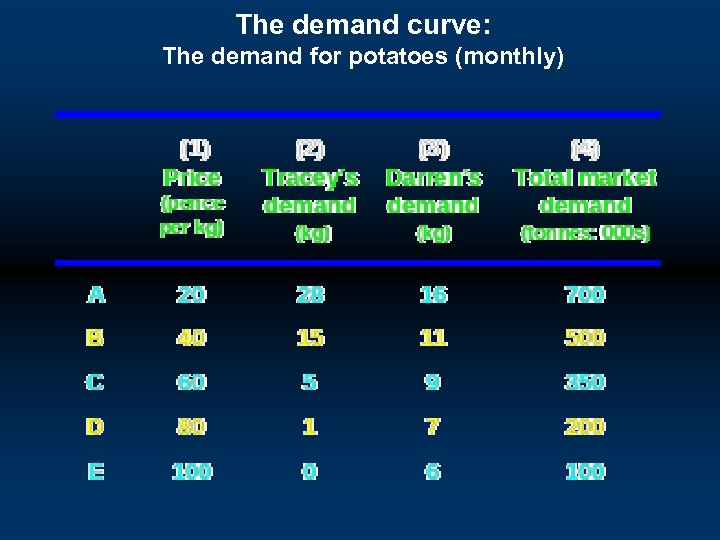The demand curve: The demand for potatoes (monthly)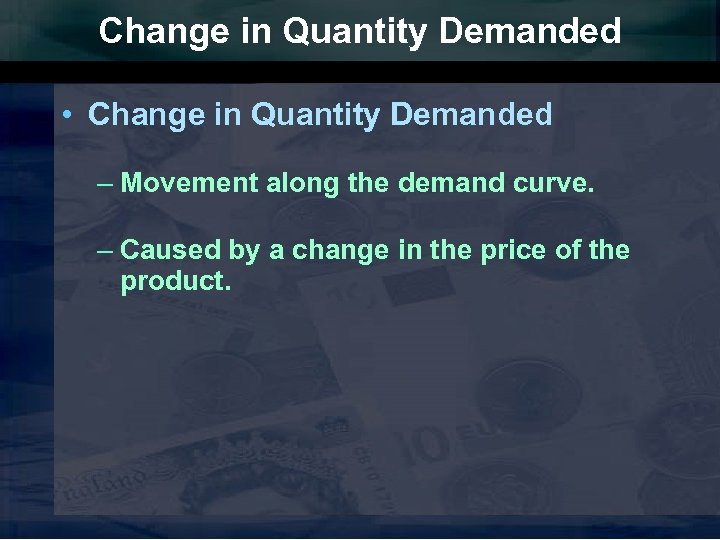Change in Quantity Demanded • Change in Quantity Demanded – Movement along the demand curve. – Caused by a change in the price of the product.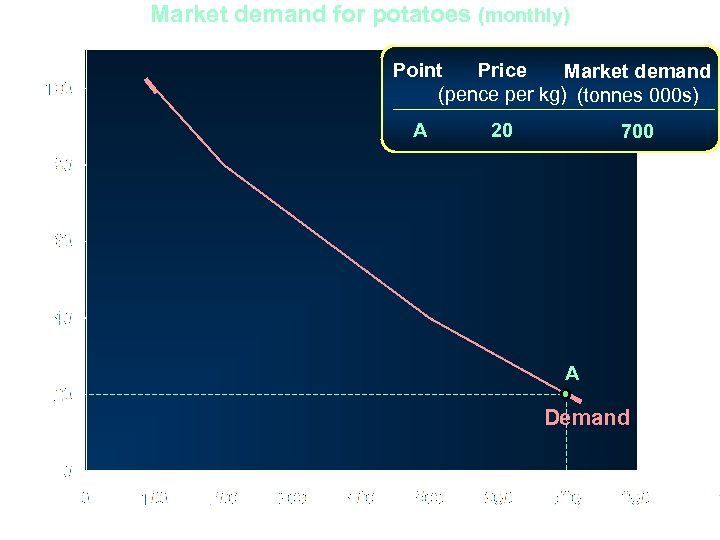Market demand for potatoes (monthly) Point Price Market demand (pence per kg) (tonnes 000 s) A 20 700 Price (pence per kg) A Demand Quantity (tonnes: 000 s)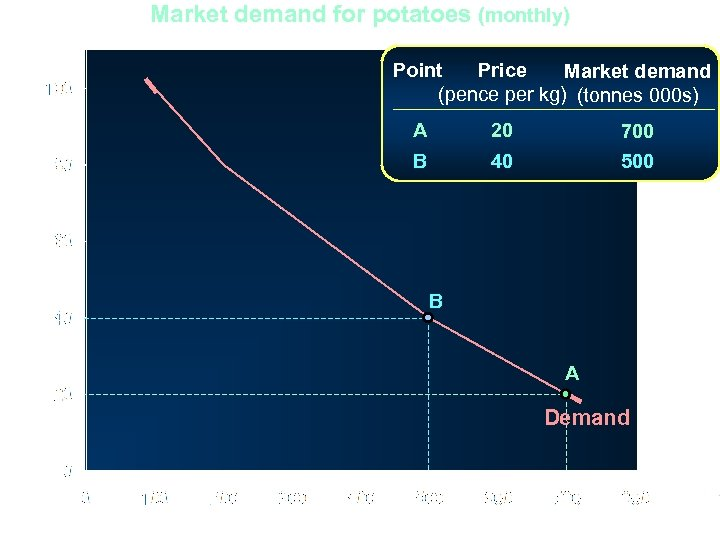Market demand for potatoes (monthly) Point Price Market demand (pence per kg) (tonnes 000 s) Price (pence per kg) A 20 700 B 40 500 B A Demand Quantity (tonnes: 000 s)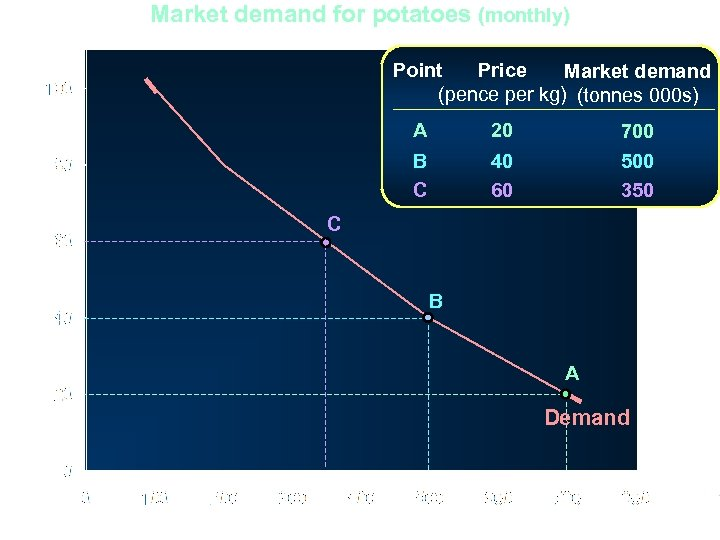Market demand for potatoes (monthly) Point Price Market demand (pence per kg) (tonnes 000 s) Price (pence per kg) A 20 700 B C 40 60 500 350 C B A Demand Quantity (tonnes: 000 s)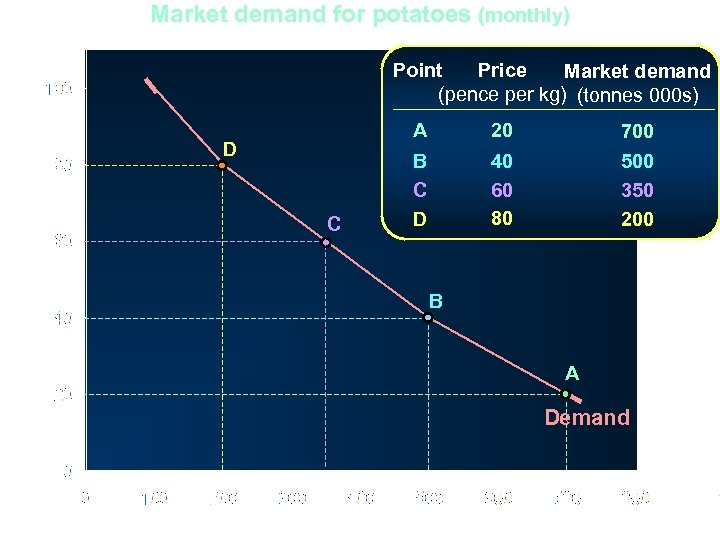Market demand for potatoes (monthly) Point Price Market demand (pence per kg) (tonnes 000 s) Price (pence per kg) A D C 20 700 B C D 40 60 80 500 350 200 B A Demand Quantity (tonnes: 000 s)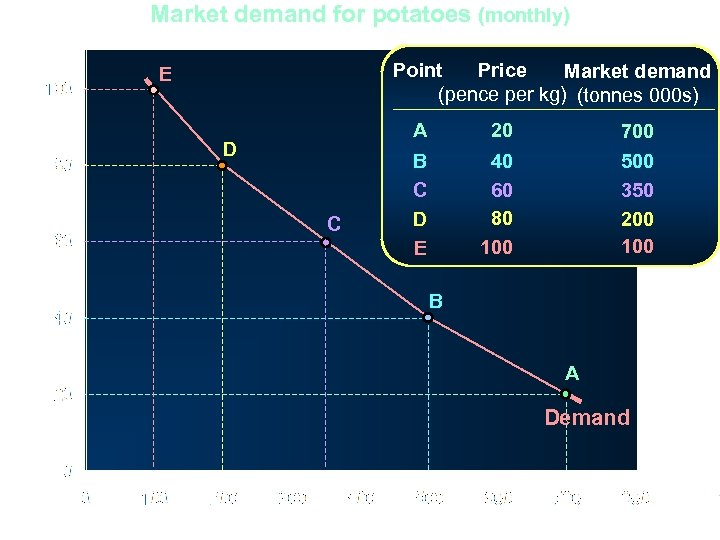Market demand for potatoes (monthly) Point Price Market demand (pence per kg) (tonnes 000 s) E Price (pence per kg) A D C 20 700 B C D E 40 60 80 100 500 350 200 100 B A Demand Quantity (tonnes: 000 s)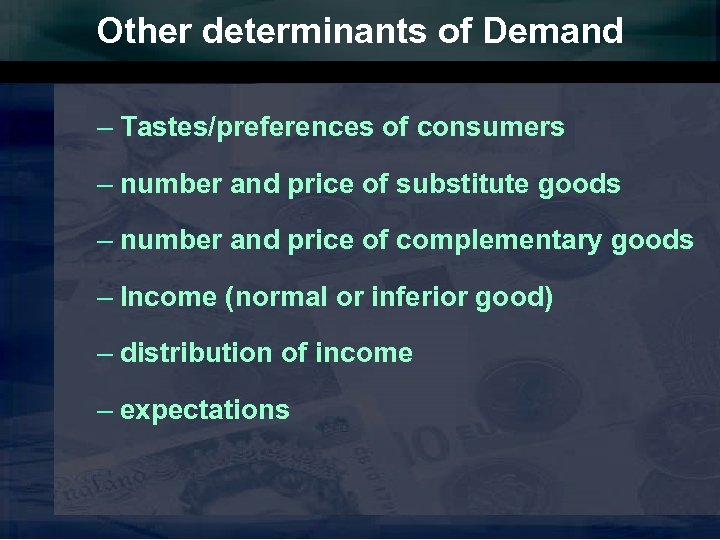Other determinants of Demand – Tastes/preferences of consumers – number and price of substitute goods – number and price of complementary goods – Income (normal or inferior good) – distribution of income – expectations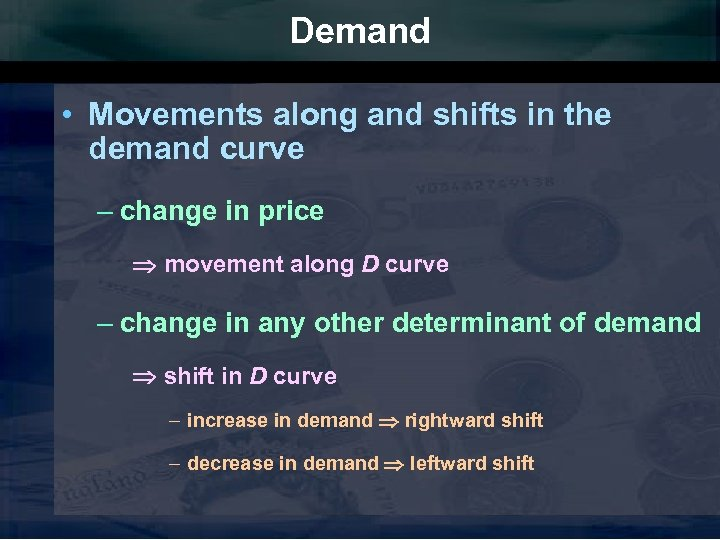Demand • Movements along and shifts in the demand curve – change in price movement along D curve – change in any other determinant of demand shift in D curve – increase in demand rightward shift – decrease in demand leftward shift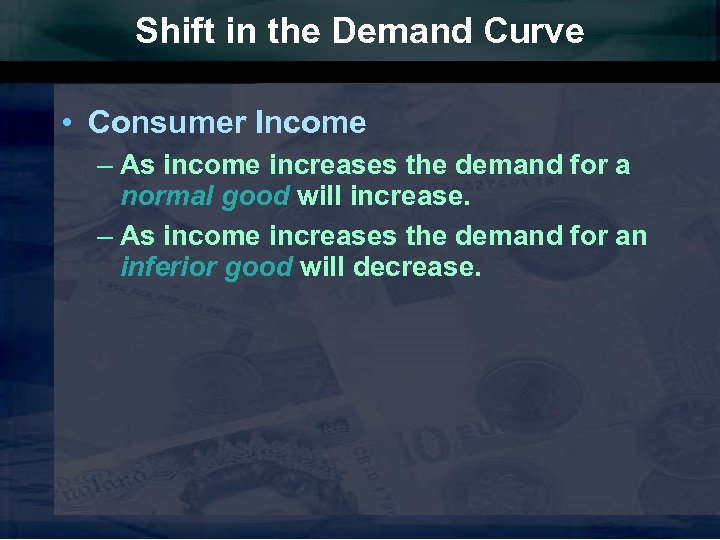Shift in the Demand Curve • Consumer Income – As income increases the demand for a normal good will increase. – As income increases the demand for an inferior good will decrease.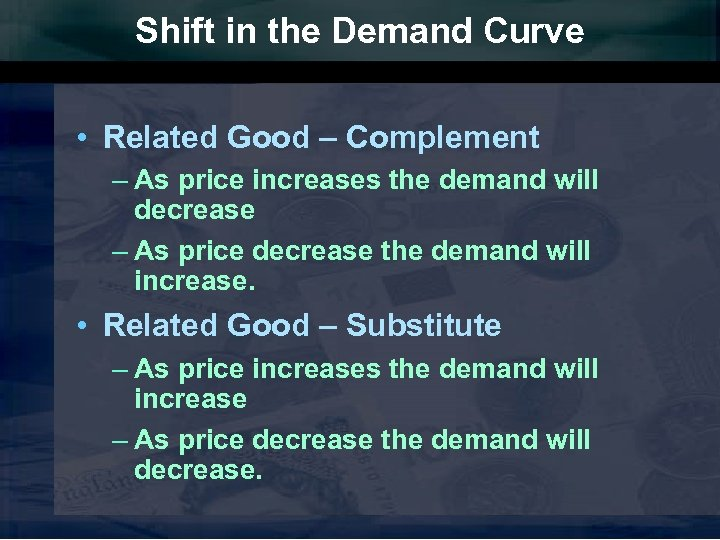Shift in the Demand Curve • Related Good – Complement – As price increases the demand will decrease – As price decrease the demand will increase. • Related Good – Substitute – As price increases the demand will increase – As price decrease the demand will decrease.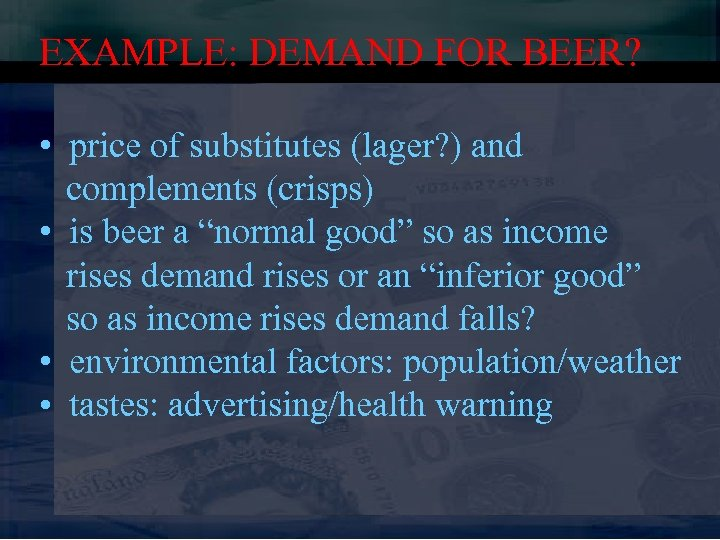EXAMPLE: DEMAND FOR BEER? • price of substitutes (lager? ) and complements (crisps) • is beer a “normal good” so as income rises demand rises or an “inferior good” so as income rises demand falls? • environmental factors: population/weather • tastes: advertising/health warning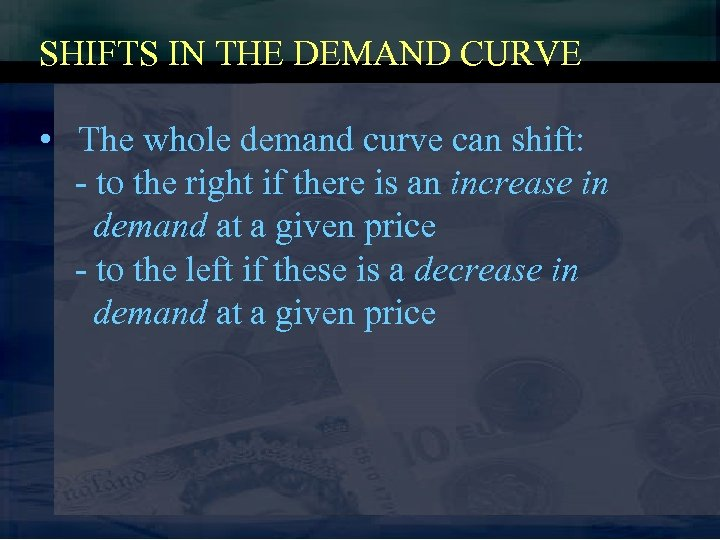SHIFTS IN THE DEMAND CURVE • The whole demand curve can shift: - to the right if there is an increase in demand at a given price - to the left if these is a decrease in demand at a given price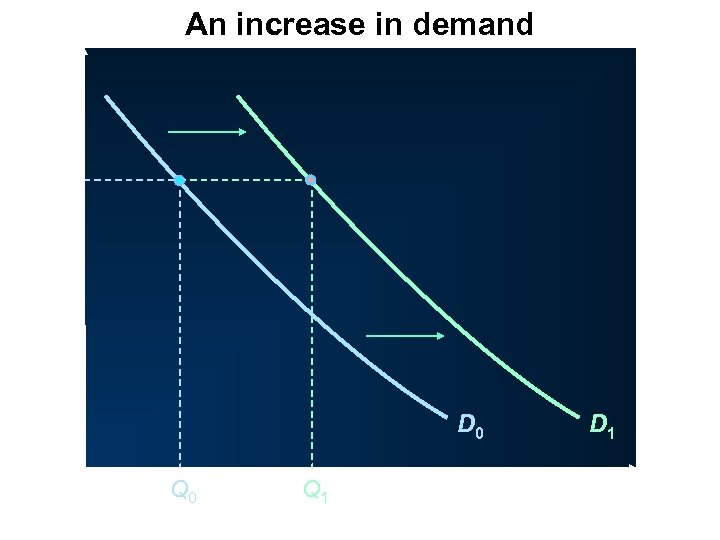An increase in demand P Price D 0 O Q 0 Q 1 Quantity D 1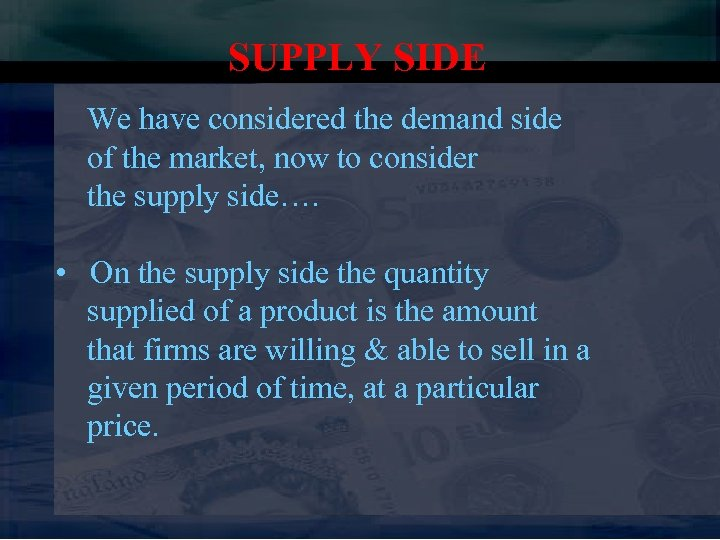SUPPLY SIDE We have considered the demand side of the market, now to consider the supply side…. • On the supply side the quantity supplied of a product is the amount that firms are willing & able to sell in a given period of time, at a particular price.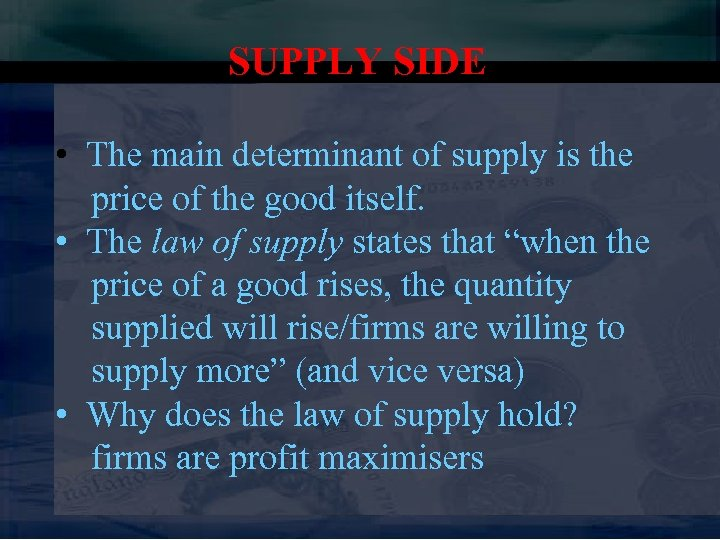SUPPLY SIDE • The main determinant of supply is the price of the good itself. • The law of supply states that “when the price of a good rises, the quantity supplied will rise/firms are willing to supply more” (and vice versa) • Why does the law of supply hold? firms are profit maximisers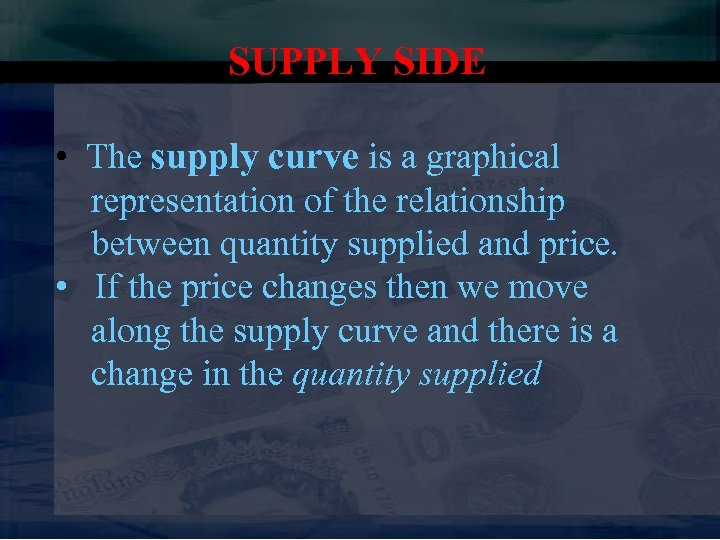SUPPLY SIDE • The supply curve is a graphical representation of the relationship between quantity supplied and price. • If the price changes then we move along the supply curve and there is a change in the quantity supplied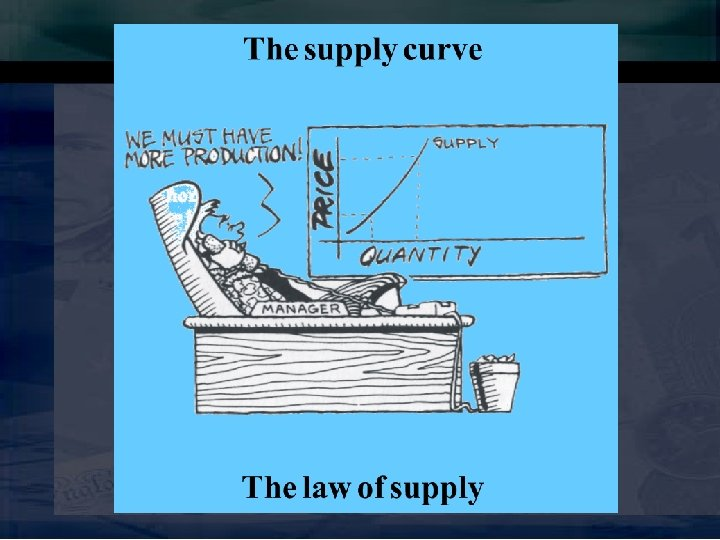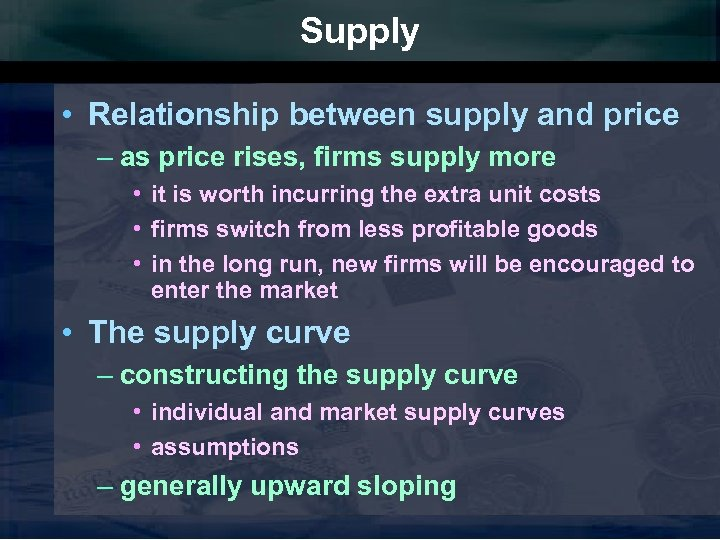Supply • Relationship between supply and price – as price rises, firms supply more • it is worth incurring the extra unit costs • firms switch from less profitable goods • in the long run, new firms will be encouraged to enter the market • The supply curve – constructing the supply curve • individual and market supply curves • assumptions – generally upward sloping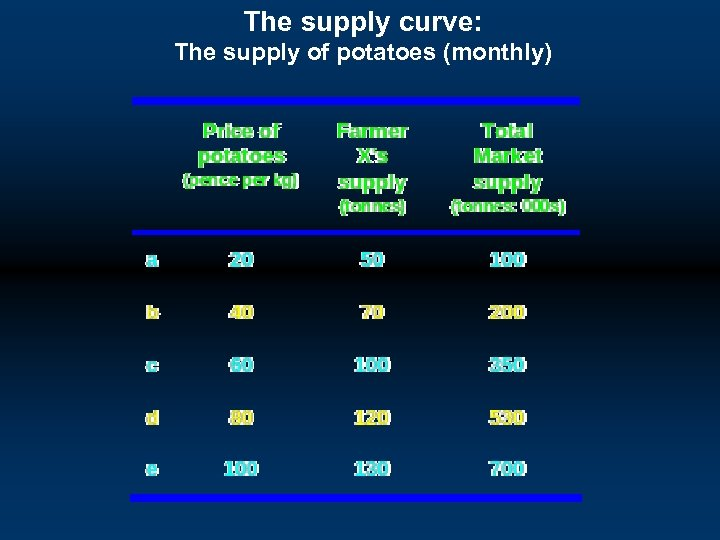The supply curve: The supply of potatoes (monthly)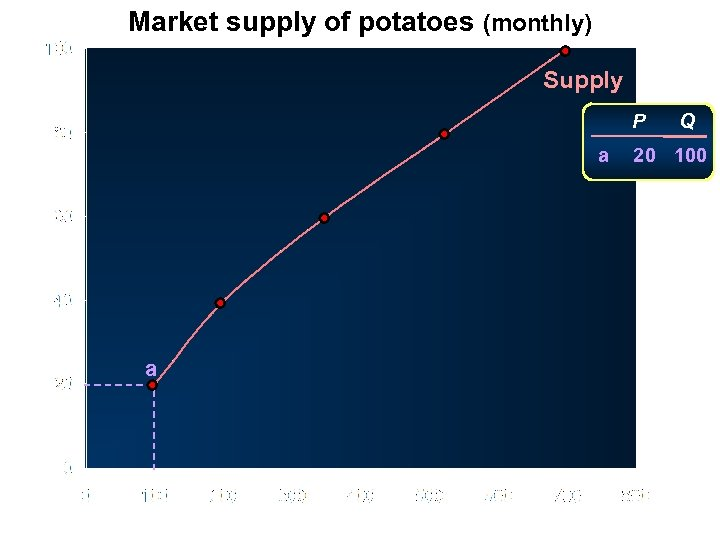Market supply of potatoes (monthly) Supply P Price (pence per kg) a a Quantity (tonnes: 000 s) Q 20 100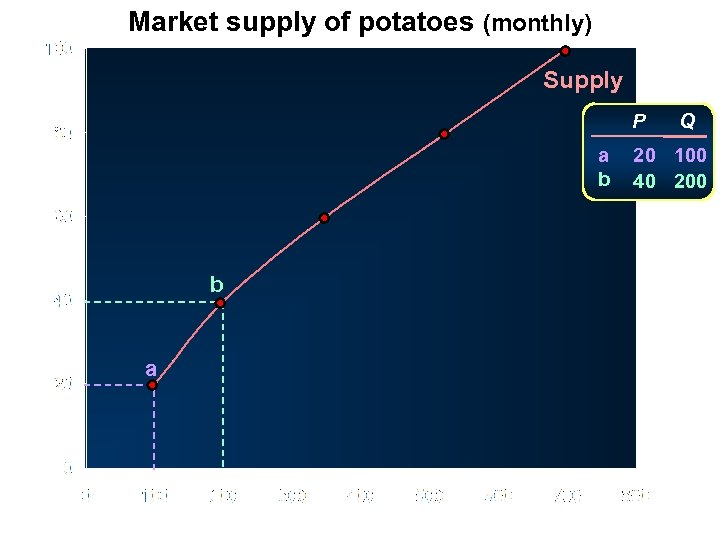Market supply of potatoes (monthly) Supply P Price (pence per kg) a b b a Quantity (tonnes: 000 s) Q 20 100 40 200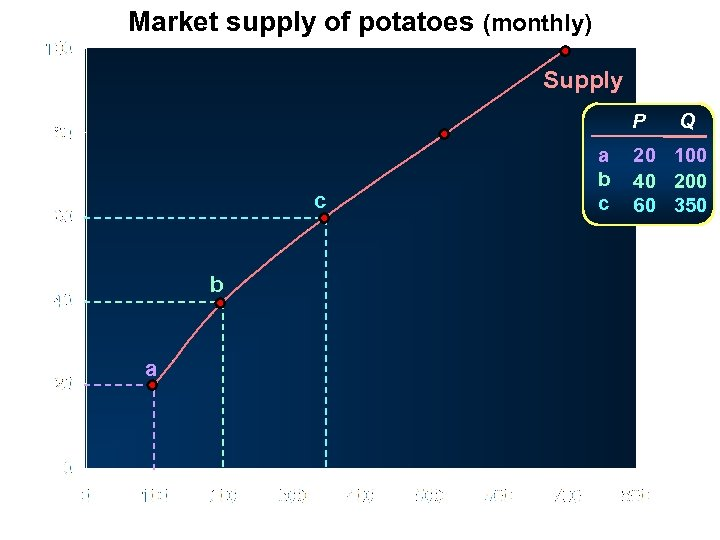Market supply of potatoes (monthly) Supply P Price (pence per kg) c b a Quantity (tonnes: 000 s) a b c Q 20 100 40 200 60 350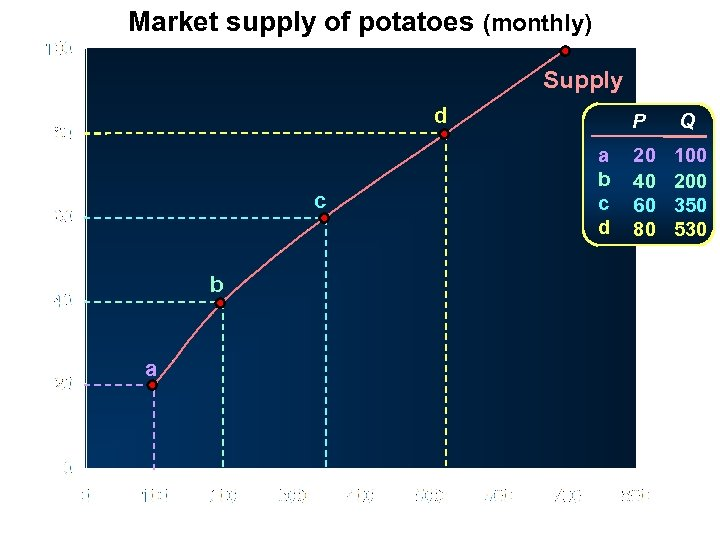Market supply of potatoes (monthly) Supply d Price (pence per kg) c b a Quantity (tonnes: 000 s) P a b c d Q 20 40 60 80 100 200 350 530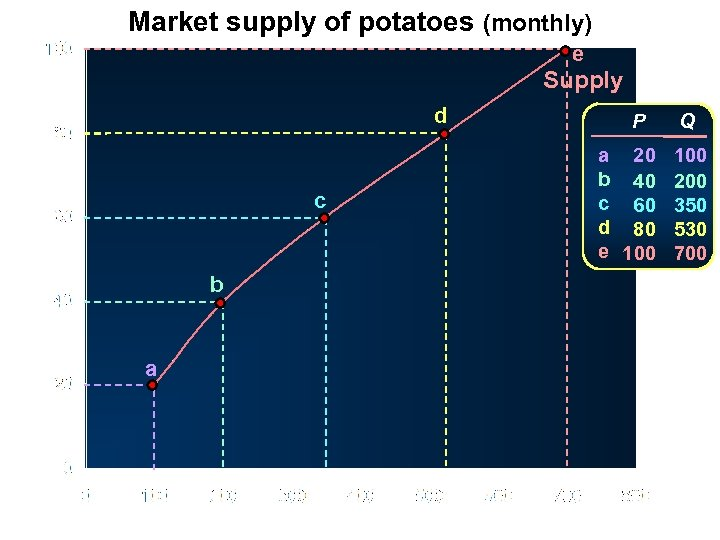Market supply of potatoes (monthly) e Supply d Price (pence per kg) c b a Quantity (tonnes: 000 s) P a 20 b 40 c 60 d 80 e 100 Q 100 200 350 530 700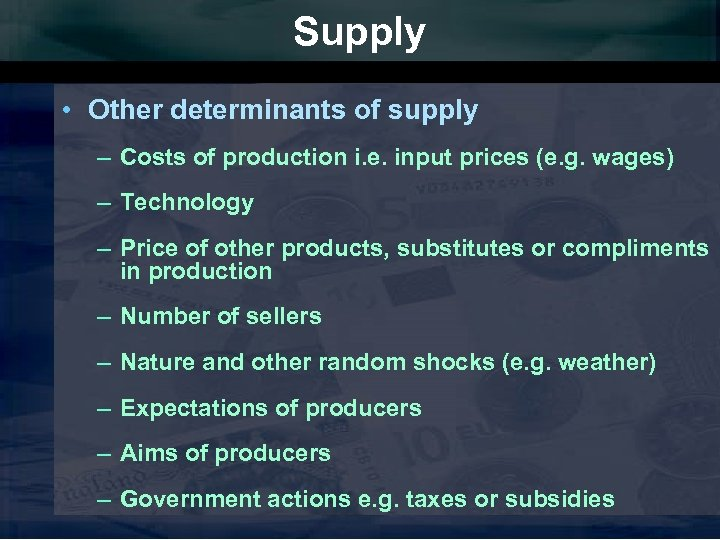Supply • Other determinants of supply – Costs of production i. e. input prices (e. g. wages) – Technology – Price of other products, substitutes or compliments in production – Number of sellers – Nature and other random shocks (e. g. weather) – Expectations of producers – Aims of producers – Government actions e. g. taxes or subsidies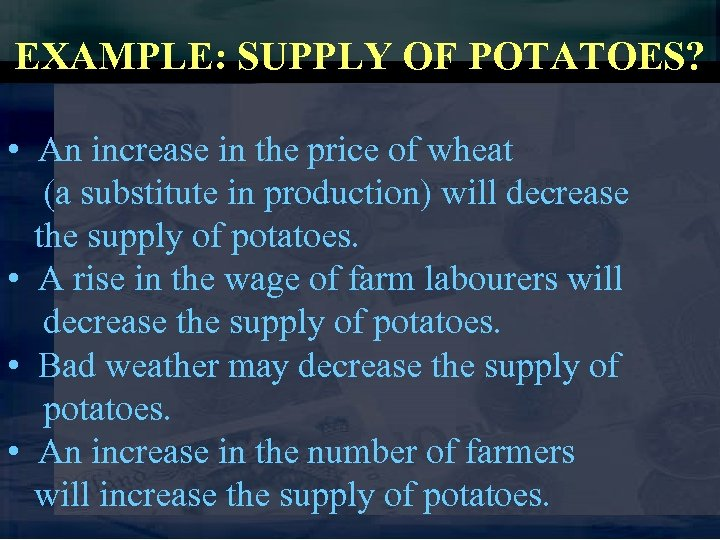EXAMPLE: SUPPLY OF POTATOES? • An increase in the price of wheat (a substitute in production) will decrease the supply of potatoes. • A rise in the wage of farm labourers will decrease the supply of potatoes. • Bad weather may decrease the supply of potatoes. • An increase in the number of farmers will increase the supply of potatoes.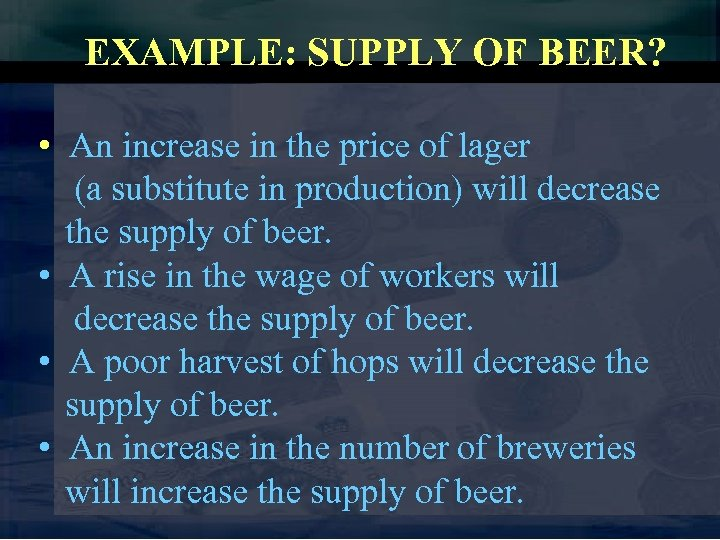EXAMPLE: SUPPLY OF BEER? • An increase in the price of lager (a substitute in production) will decrease the supply of beer. • A rise in the wage of workers will decrease the supply of beer. • A poor harvest of hops will decrease the supply of beer. • An increase in the number of breweries will increase the supply of beer.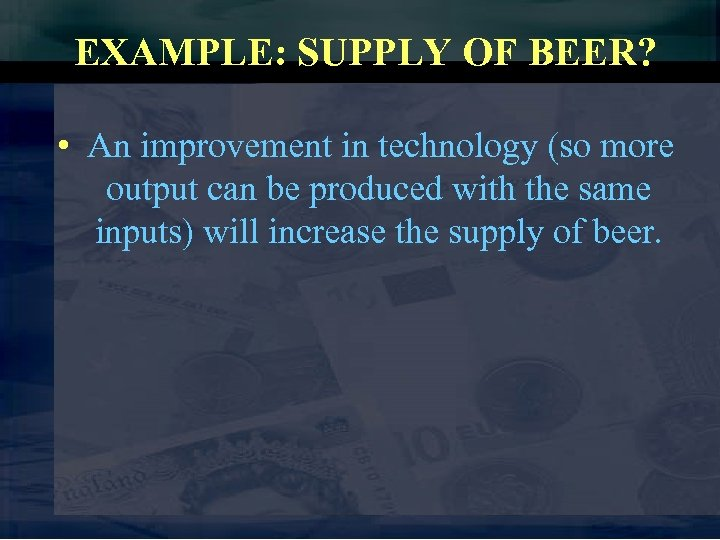EXAMPLE: SUPPLY OF BEER? • An improvement in technology (so more output can be produced with the same inputs) will increase the supply of beer.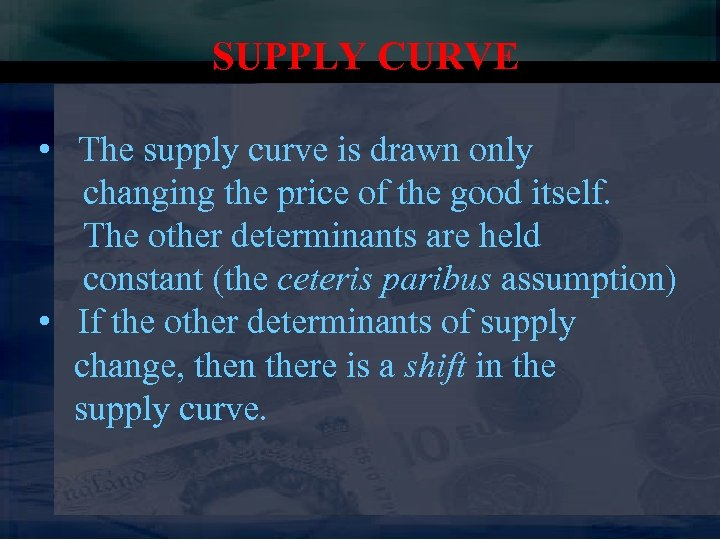SUPPLY CURVE • The supply curve is drawn only changing the price of the good itself. The other determinants are held constant (the ceteris paribus assumption) • If the other determinants of supply change, then there is a shift in the supply curve.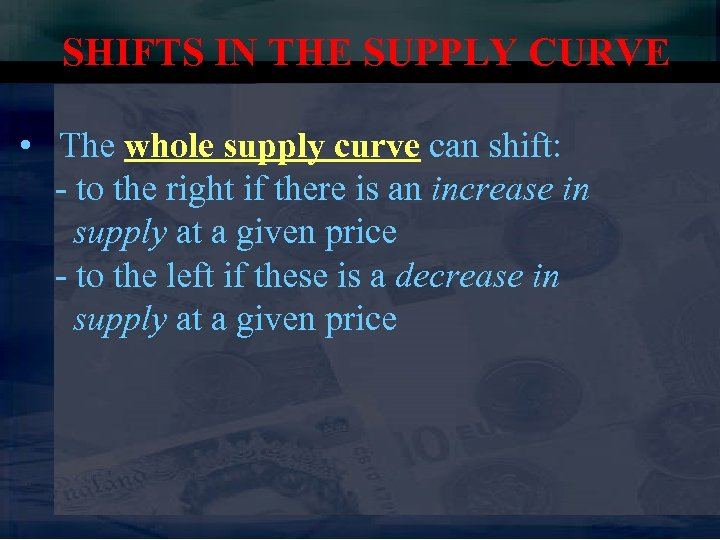SHIFTS IN THE SUPPLY CURVE • The whole supply curve can shift: - to the right if there is an increase in supply at a given price - to the left if these is a decrease in supply at a given price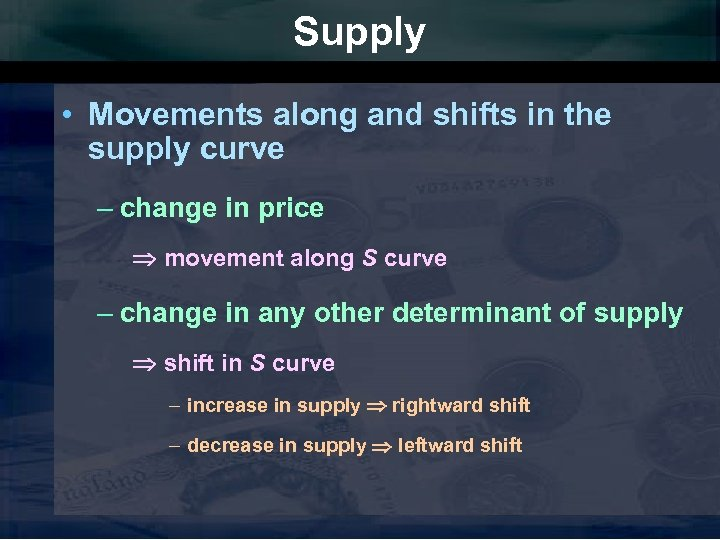Supply • Movements along and shifts in the supply curve – change in price movement along S curve – change in any other determinant of supply shift in S curve – increase in supply rightward shift – decrease in supply leftward shift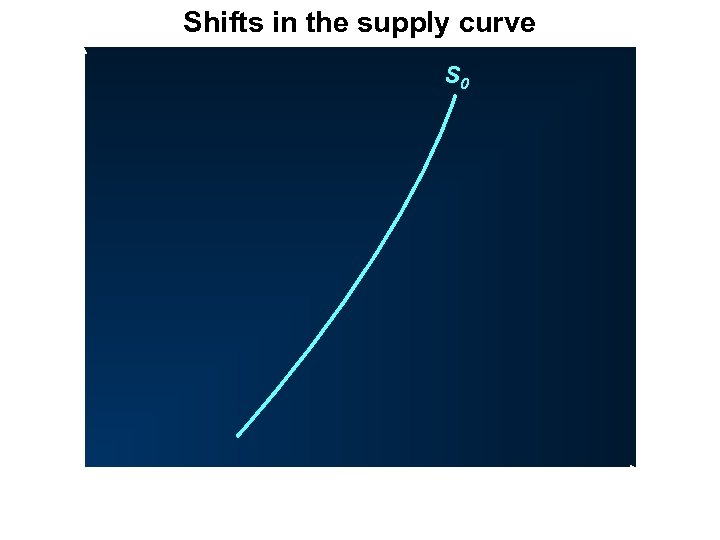Shifts in the supply curve P O S 0 Q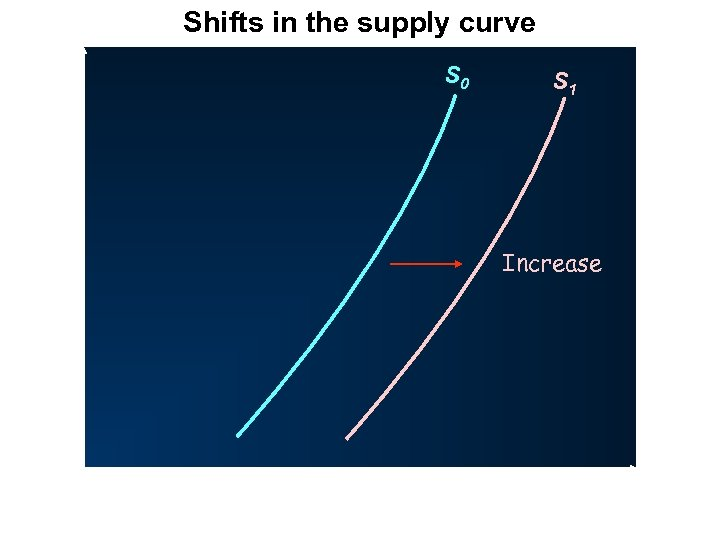Shifts in the supply curve P S 0 S 1 Increase O Q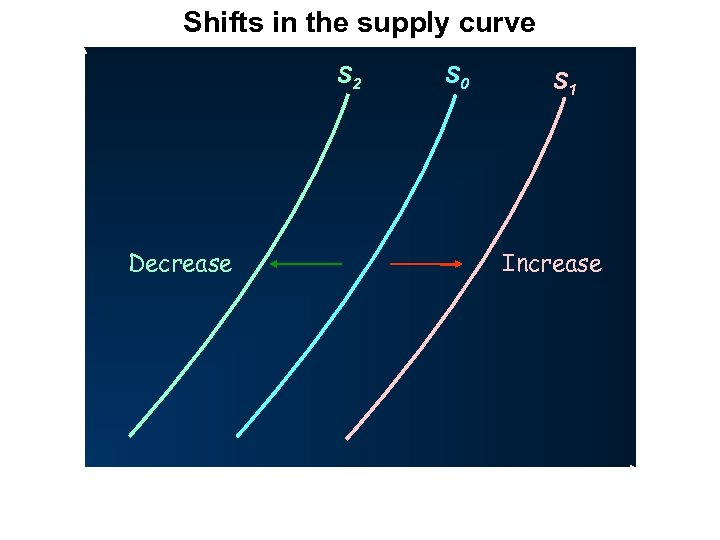Shifts in the supply curve P S 2 Decrease O S 0 S 1 Increase Q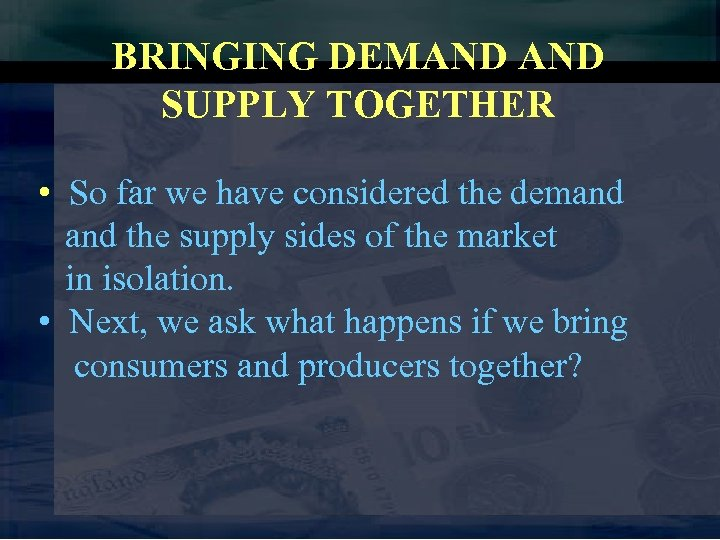BRINGING DEMAND SUPPLY TOGETHER • So far we have considered the demand the supply sides of the market in isolation. • Next, we ask what happens if we bring consumers and producers together?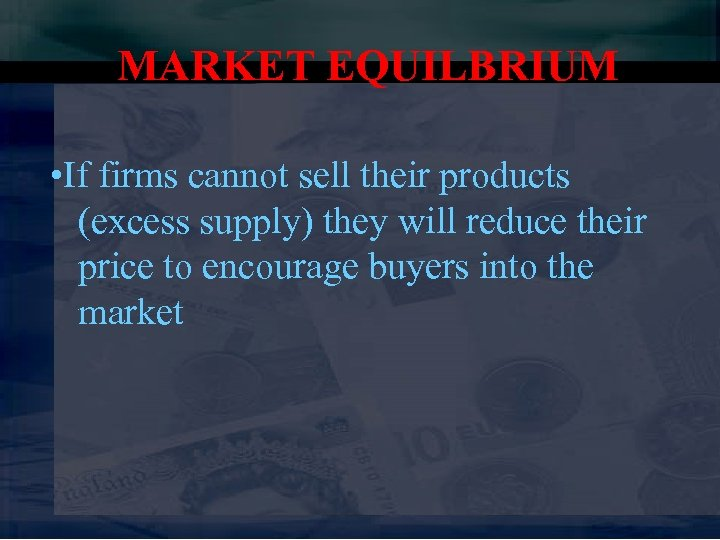MARKET EQUILBRIUM • If firms cannot sell their products (excess supply) they will reduce their price to encourage buyers into the market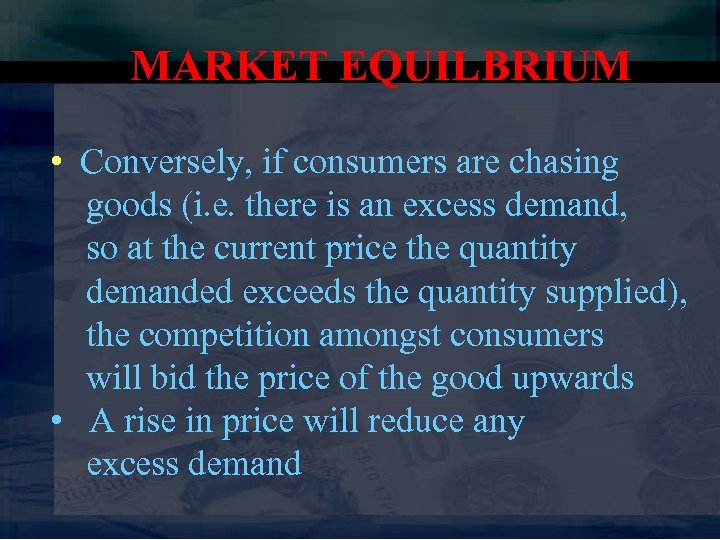MARKET EQUILBRIUM • Conversely, if consumers are chasing goods (i. e. there is an excess demand, so at the current price the quantity demanded exceeds the quantity supplied), the competition amongst consumers will bid the price of the good upwards • A rise in price will reduce any excess demand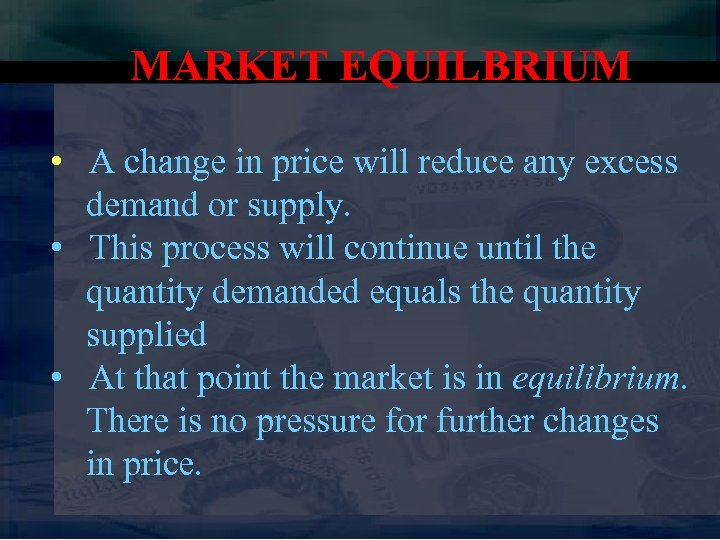MARKET EQUILBRIUM • A change in price will reduce any excess demand or supply. • This process will continue until the quantity demanded equals the quantity supplied • At that point the market is in equilibrium. There is no pressure for further changes in price.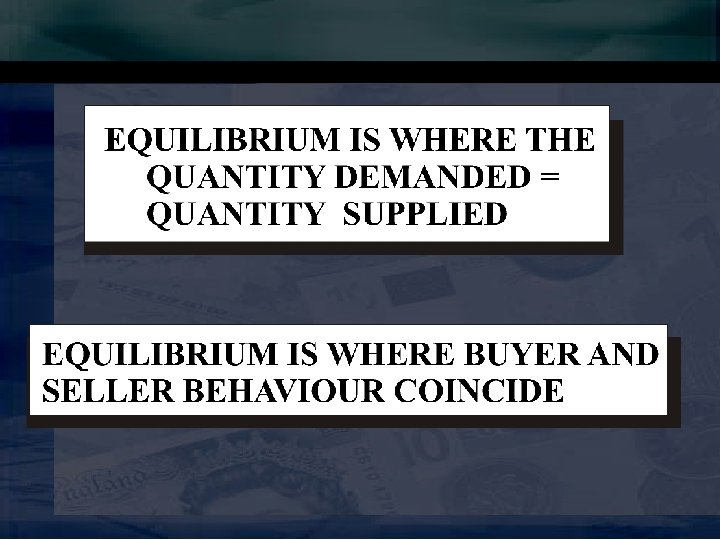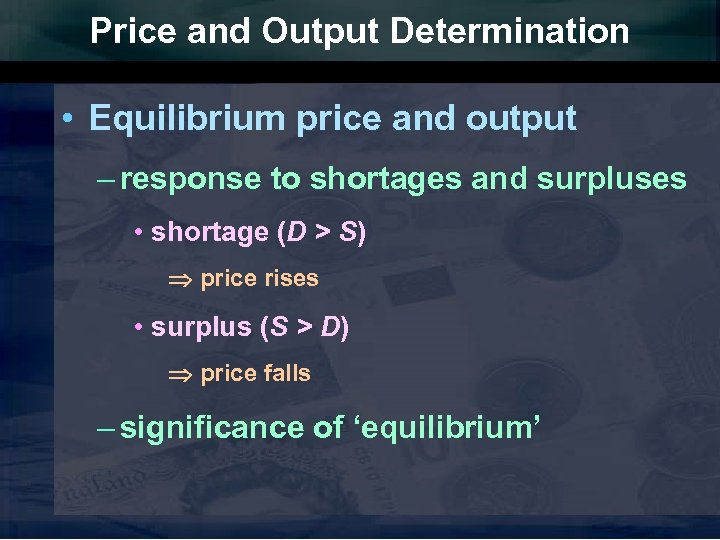Price and Output Determination • Equilibrium price and output – response to shortages and surpluses • shortage (D > S) price rises • surplus (S > D) price falls – significance of ‘equilibrium’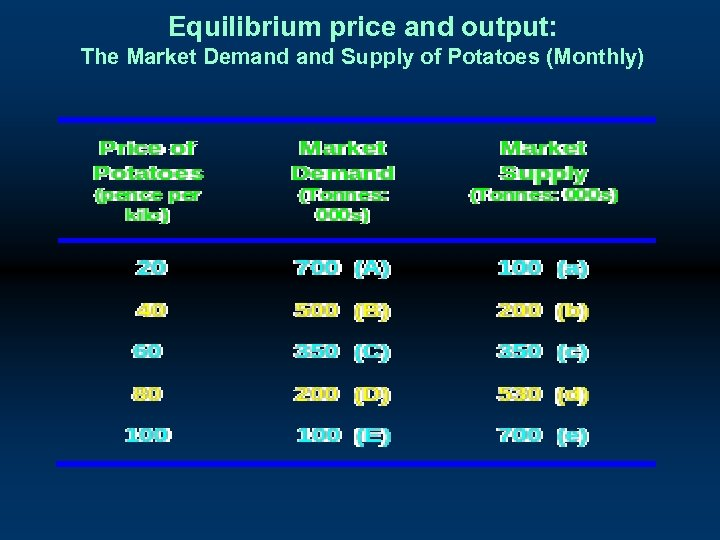Equilibrium price and output: The Market Demand Supply of Potatoes (Monthly)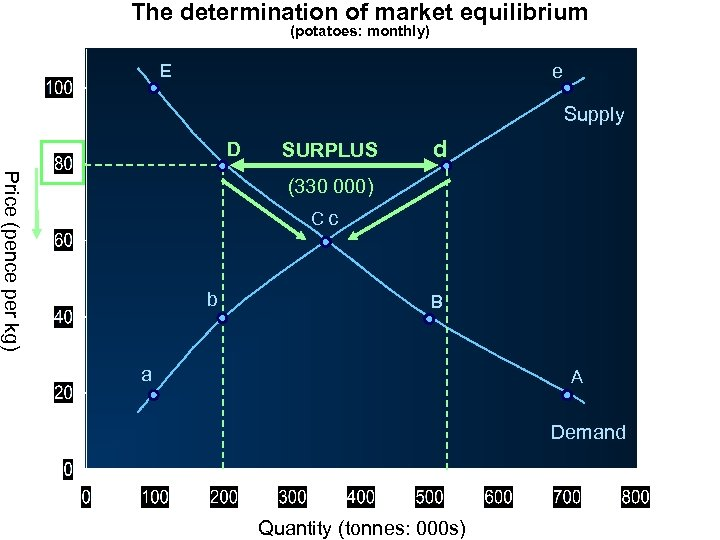The determination of market equilibrium (potatoes: monthly) E e Supply D SURPLUS d Price (pence per kg) (330 000) Cc b B a A Demand Quantity (tonnes: 000 s)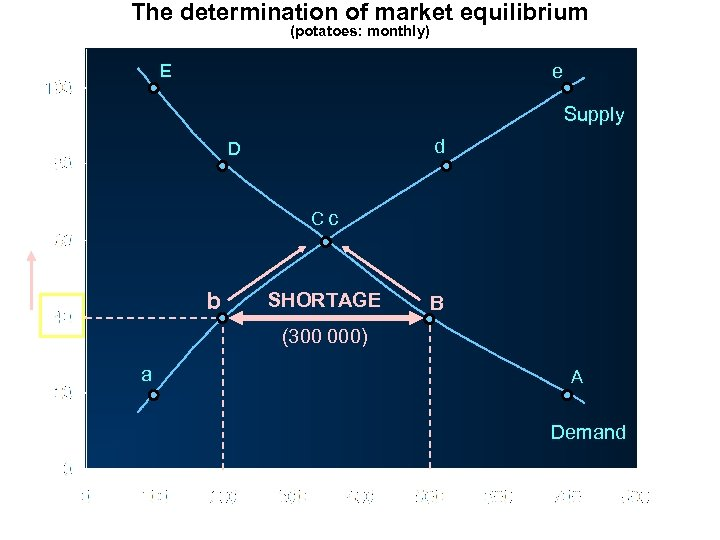The determination of market equilibrium (potatoes: monthly) E e Supply d D Price (pence per kg) Cc b SHORTAGE B (300 000) a A Demand Quantity (tonnes: 000 s)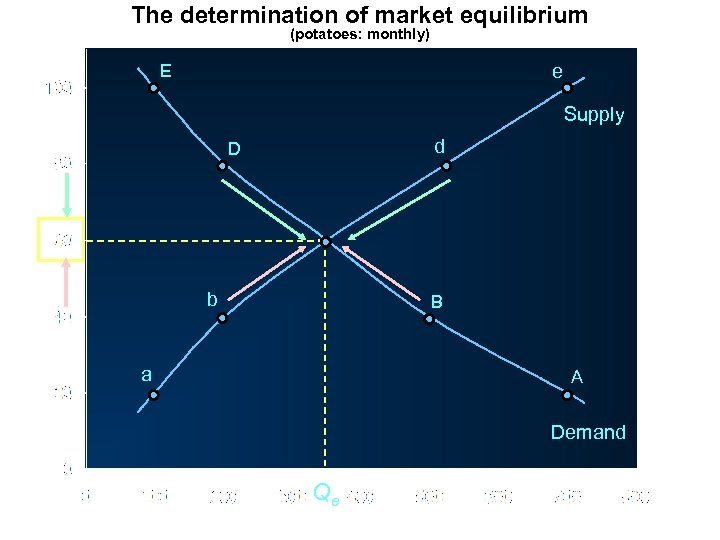The determination of market equilibrium (potatoes: monthly) E e Supply d D Price (pence per kg) b B a A Demand Qe Quantity (tonnes: 000 s)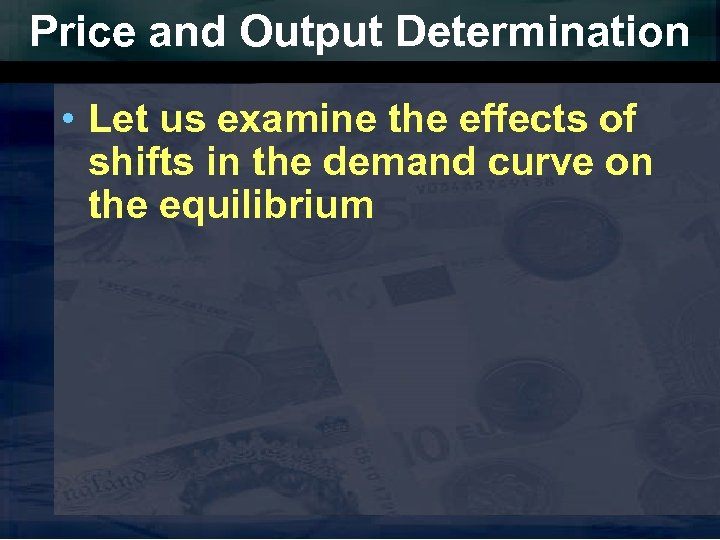Price and Output Determination • Let us examine the effects of shifts in the demand curve on the equilibrium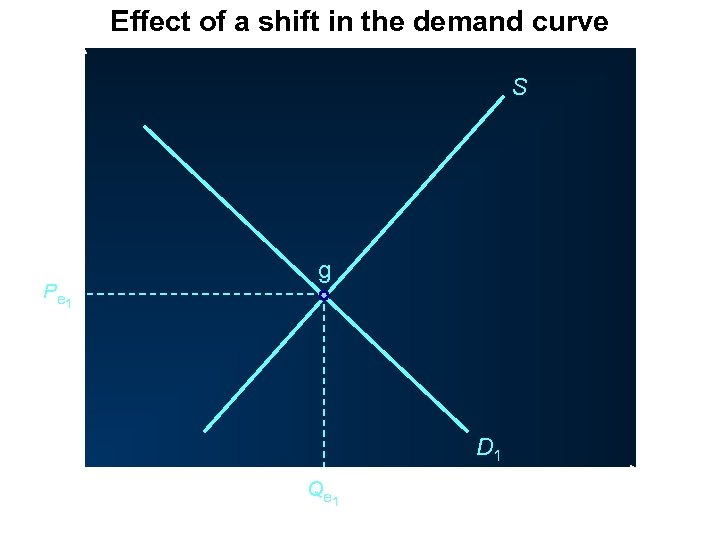Effect of a shift in the demand curve P S P e 1 g D 1 O Q e 1 Q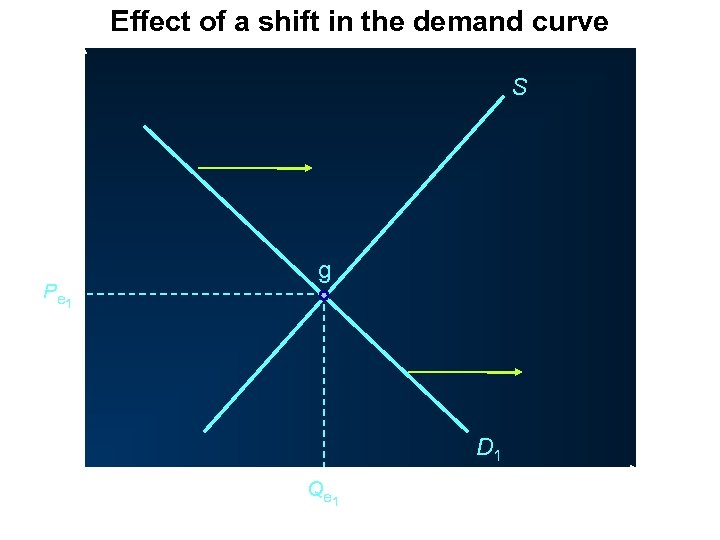Effect of a shift in the demand curve P S P e 1 g D 1 O Q e 1 Q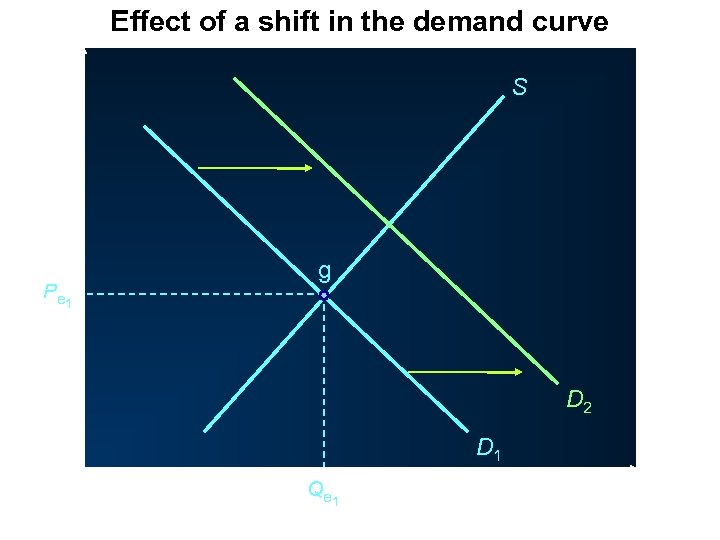Effect of a shift in the demand curve P S P e 1 g D 2 D 1 O Q e 1 Q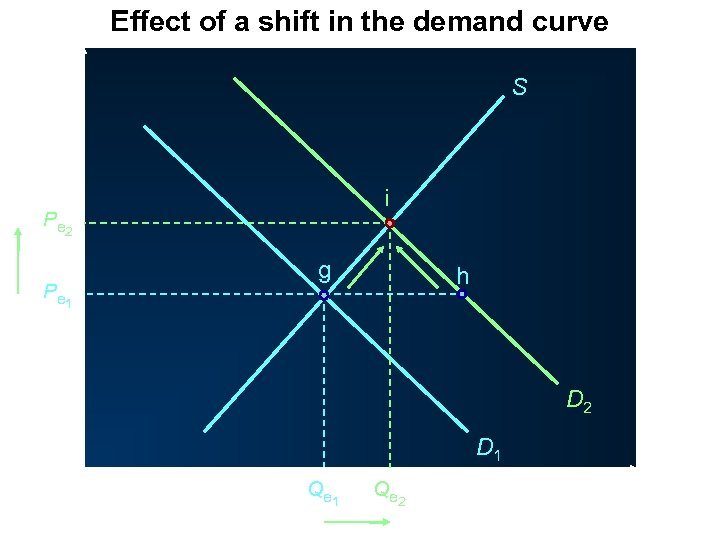Effect of a shift in the demand curve P S i P e 2 P e 1 g h D 2 D 1 O Q e 1 Q e 2 Q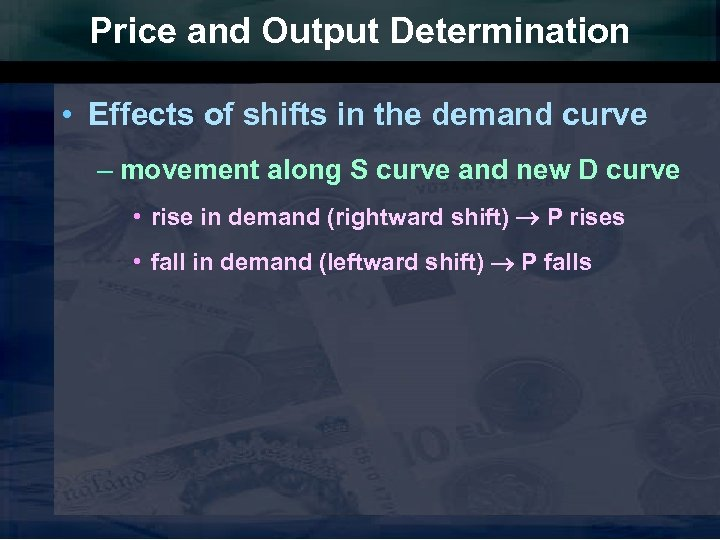Price and Output Determination • Effects of shifts in the demand curve – movement along S curve and new D curve • rise in demand (rightward shift) P rises • fall in demand (leftward shift) P falls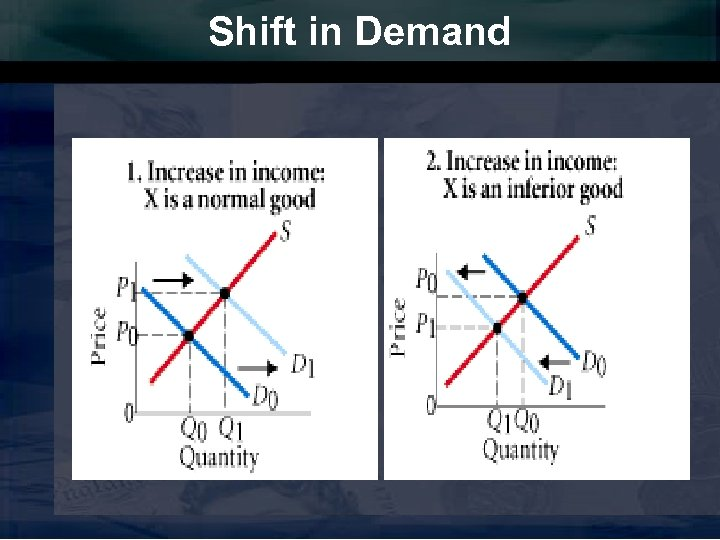Shift in Demand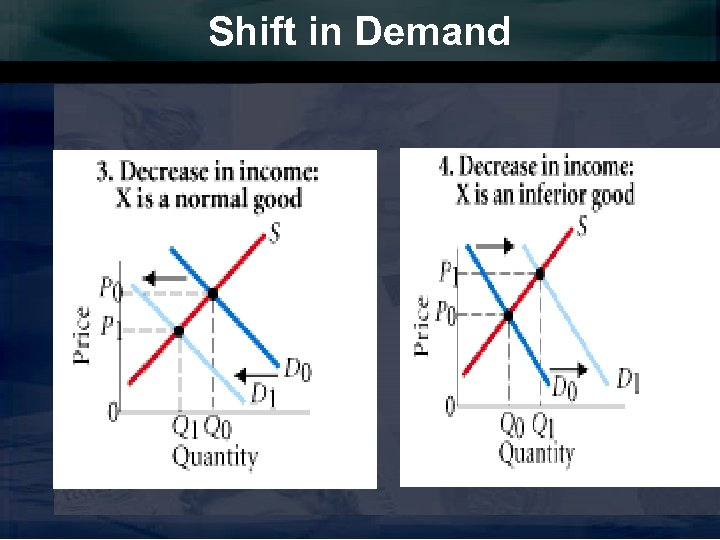Shift in Demand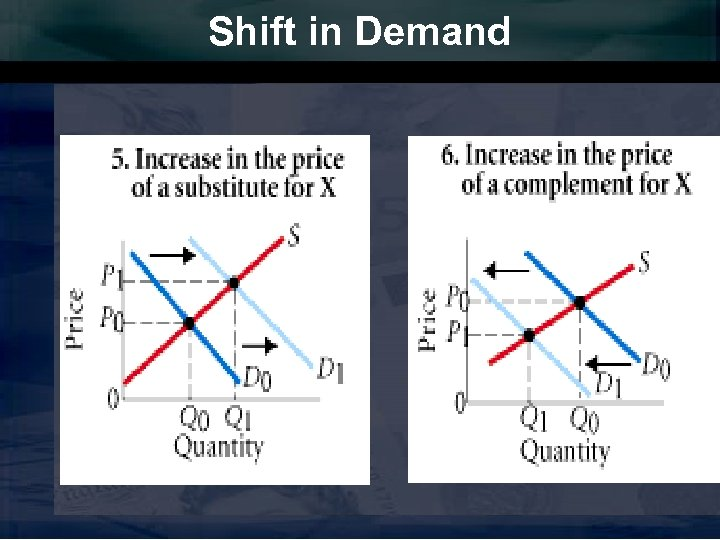Shift in Demand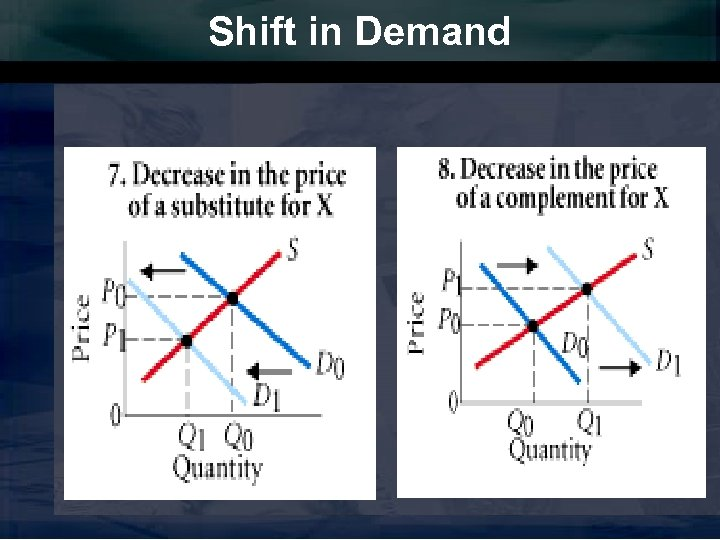Shift in Demand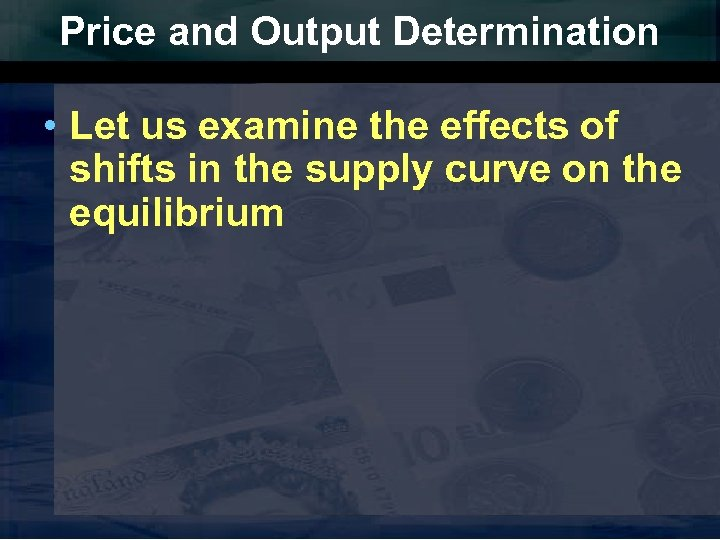Price and Output Determination • Let us examine the effects of shifts in the supply curve on the equilibrium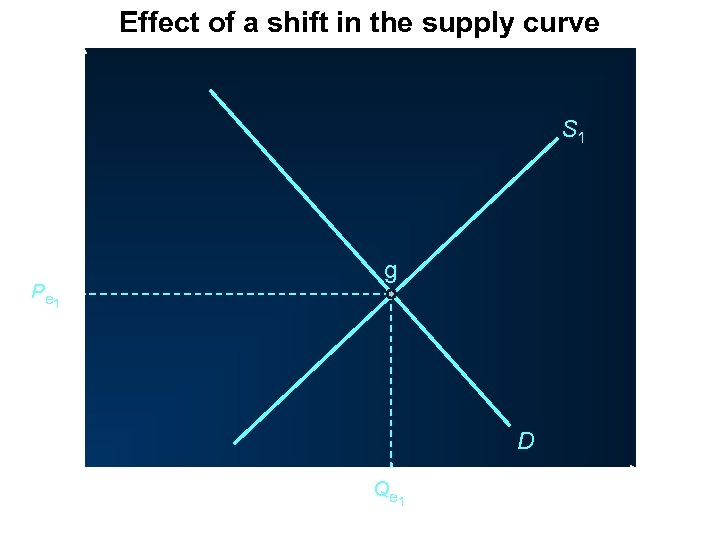Effect of a shift in the supply curve P S 1 g P e 1 D O Q e 1 Q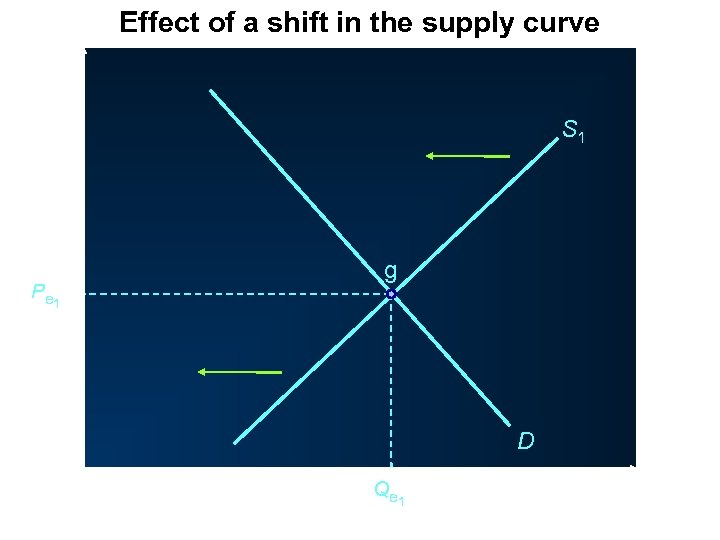Effect of a shift in the supply curve P S 1 g P e 1 D O Q e 1 Q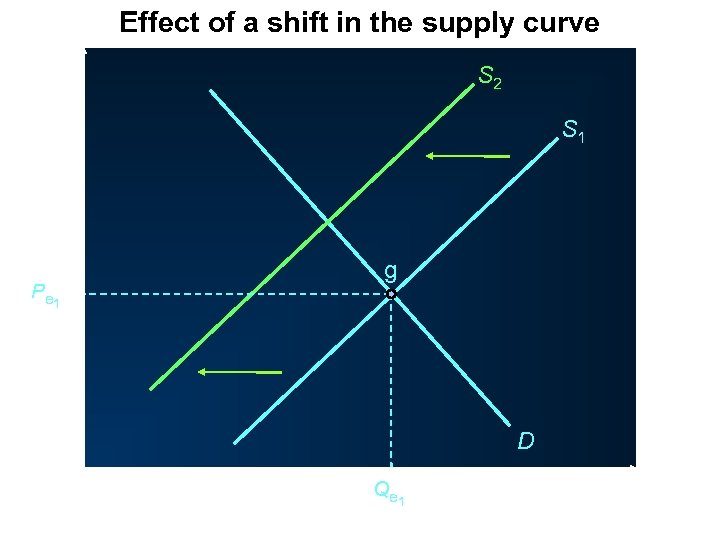Effect of a shift in the supply curve P S 2 S 1 g P e 1 D O Q e 1 Q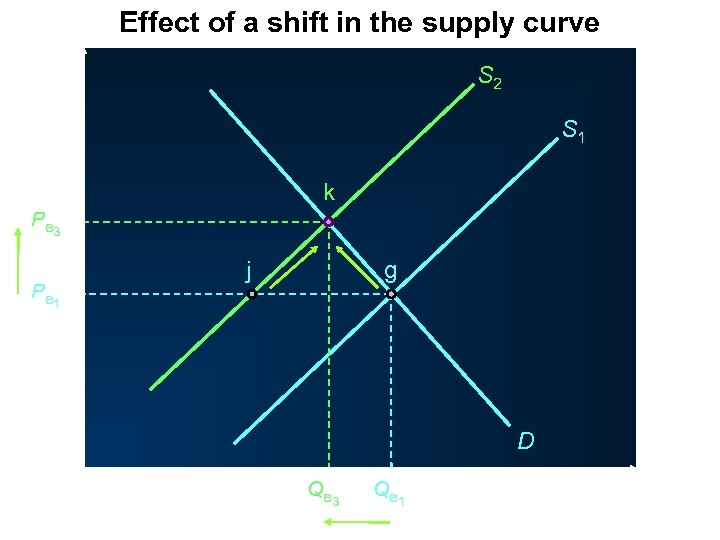Effect of a shift in the supply curve P S 2 S 1 k P e 3 j P e 1 g D O Q e 3 Q e 1 Q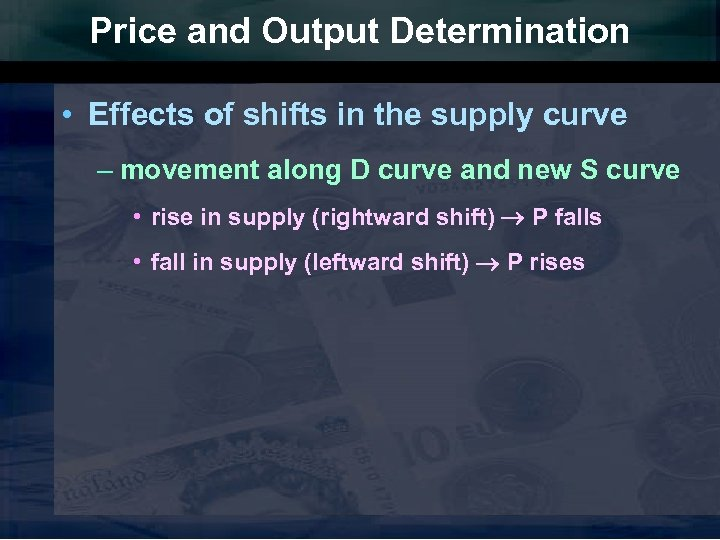Price and Output Determination • Effects of shifts in the supply curve – movement along D curve and new S curve • rise in supply (rightward shift) P falls • fall in supply (leftward shift) P rises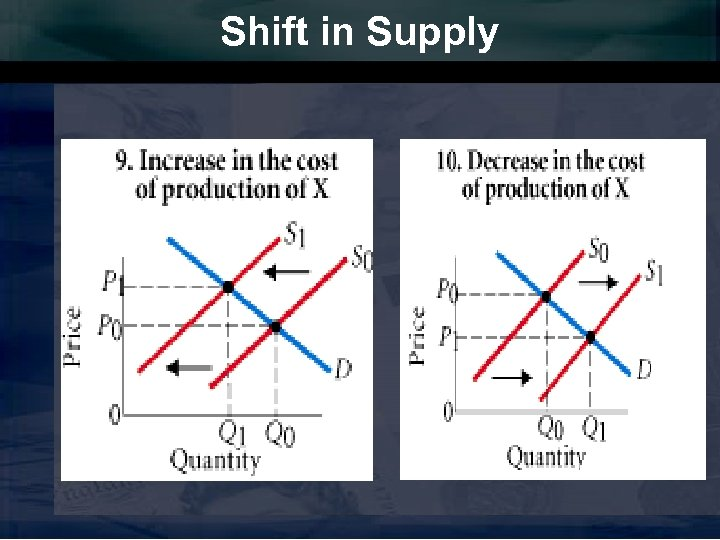Shift in Supply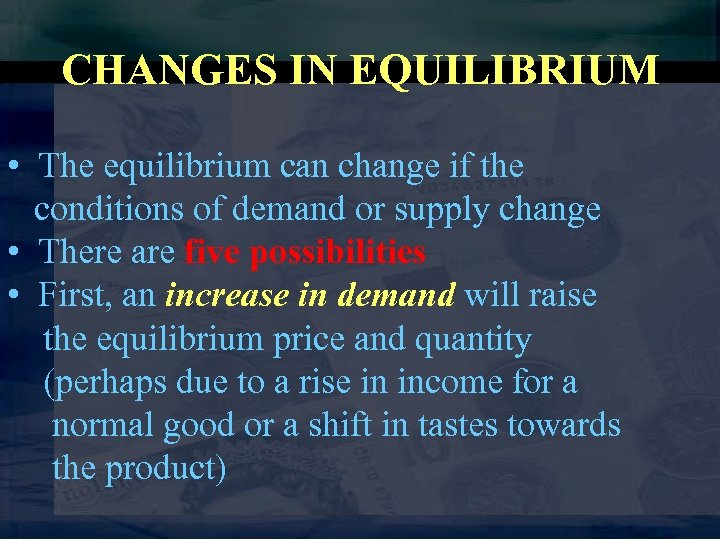CHANGES IN EQUILIBRIUM • The equilibrium can change if the conditions of demand or supply change • There are five possibilities • First, an increase in demand will raise the equilibrium price and quantity (perhaps due to a rise in income for a normal good or a shift in tastes towards the product)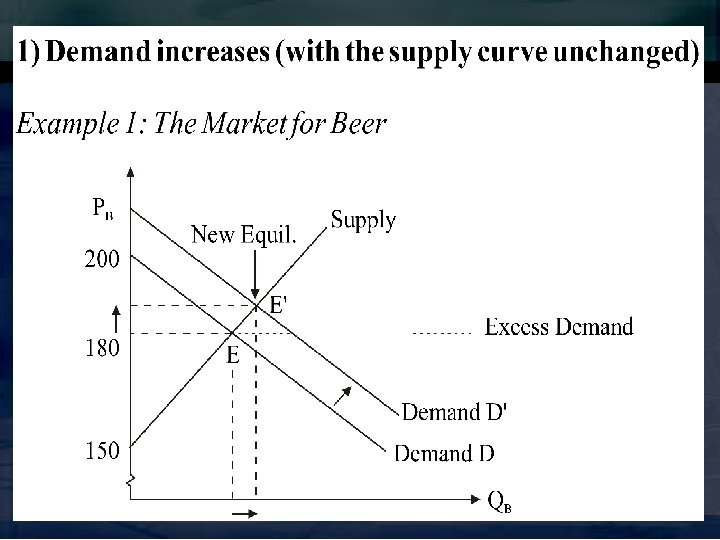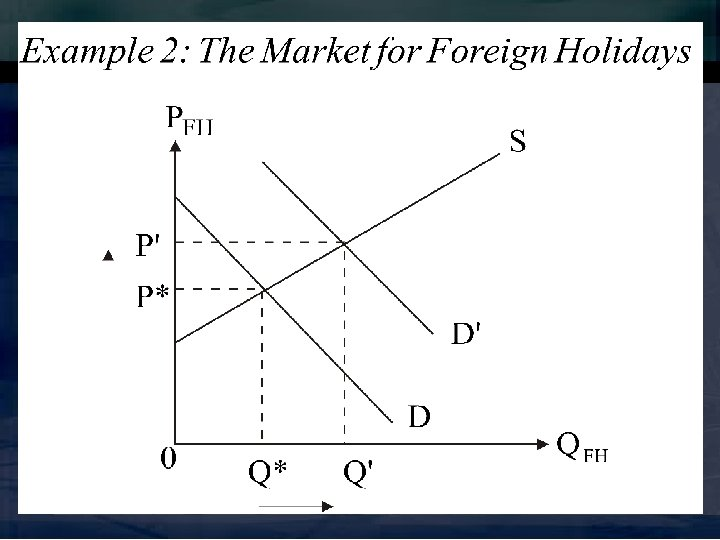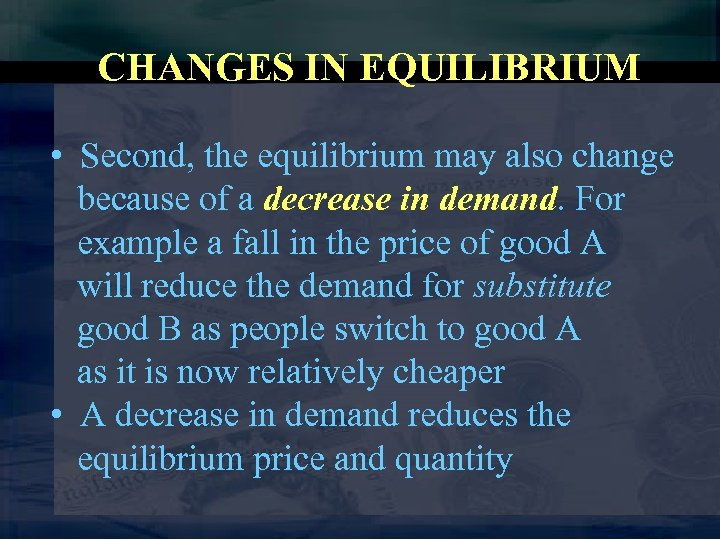CHANGES IN EQUILIBRIUM • Second, the equilibrium may also change because of a decrease in demand. For example a fall in the price of good A will reduce the demand for substitute good B as people switch to good A as it is now relatively cheaper • A decrease in demand reduces the equilibrium price and quantity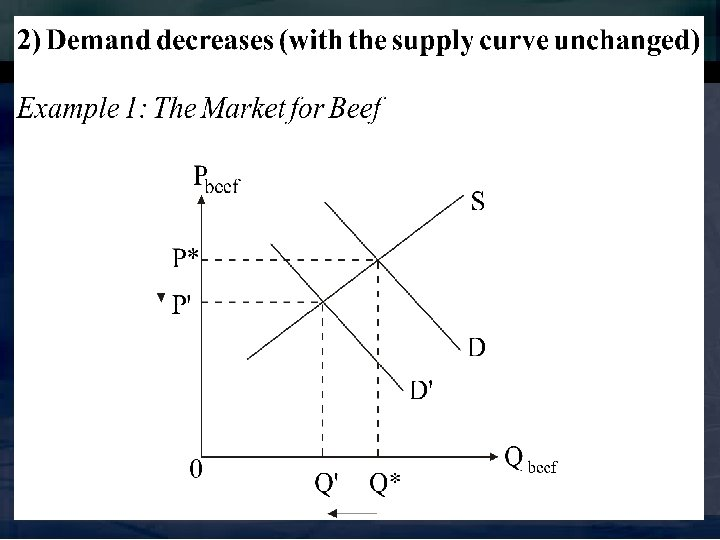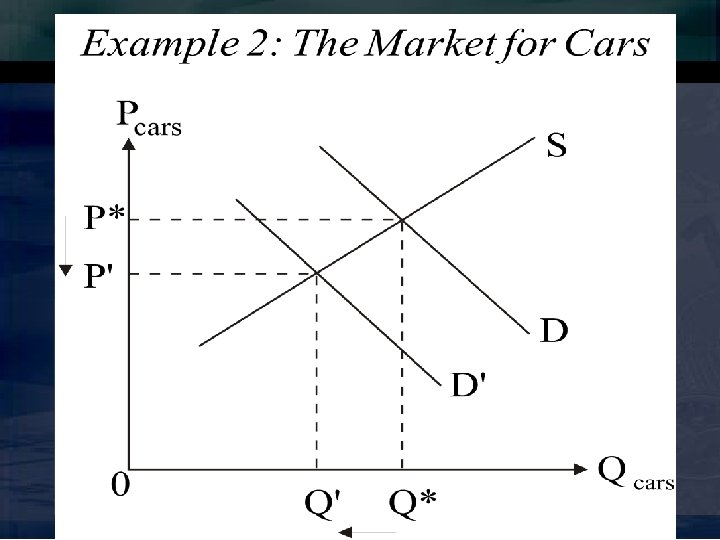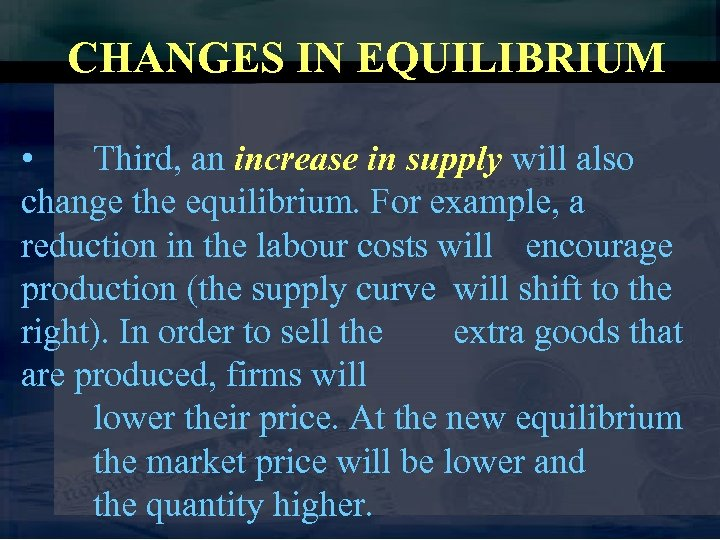CHANGES IN EQUILIBRIUM • Third, an increase in supply will also change the equilibrium. For example, a reduction in the labour costs will encourage production (the supply curve will shift to the right). In order to sell the extra goods that are produced, firms will lower their price. At the new equilibrium the market price will be lower and the quantity higher.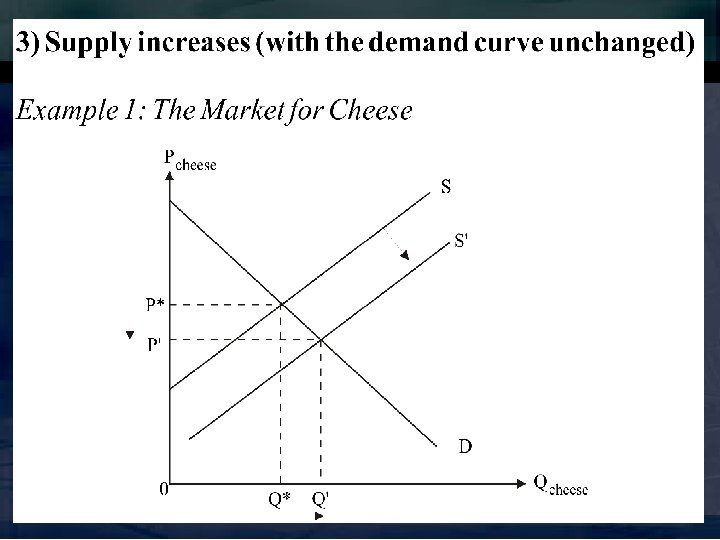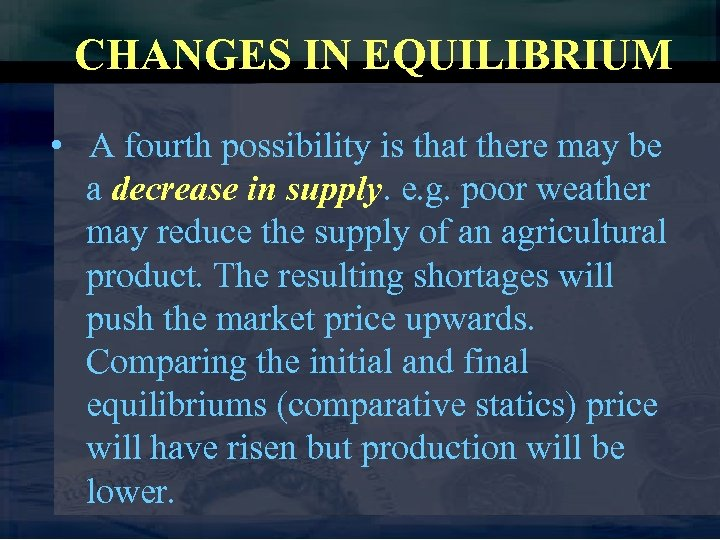CHANGES IN EQUILIBRIUM • A fourth possibility is that there may be a decrease in supply. e. g. poor weather may reduce the supply of an agricultural product. The resulting shortages will push the market price upwards. Comparing the initial and final equilibriums (comparative statics) price will have risen but production will be lower.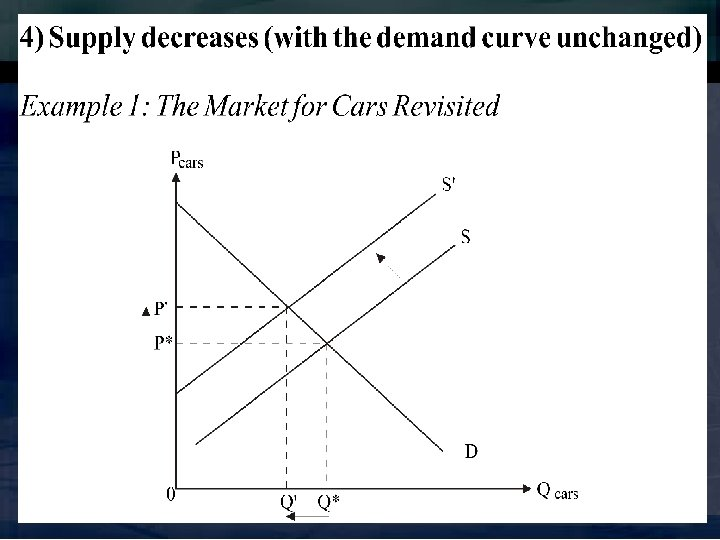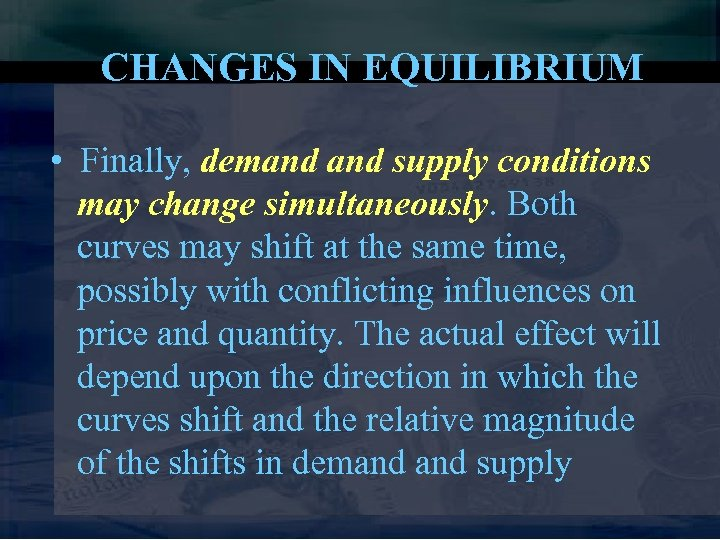CHANGES IN EQUILIBRIUM • Finally, demand supply conditions may change simultaneously. Both curves may shift at the same time, possibly with conflicting influences on price and quantity. The actual effect will depend upon the direction in which the curves shift and the relative magnitude of the shifts in demand supply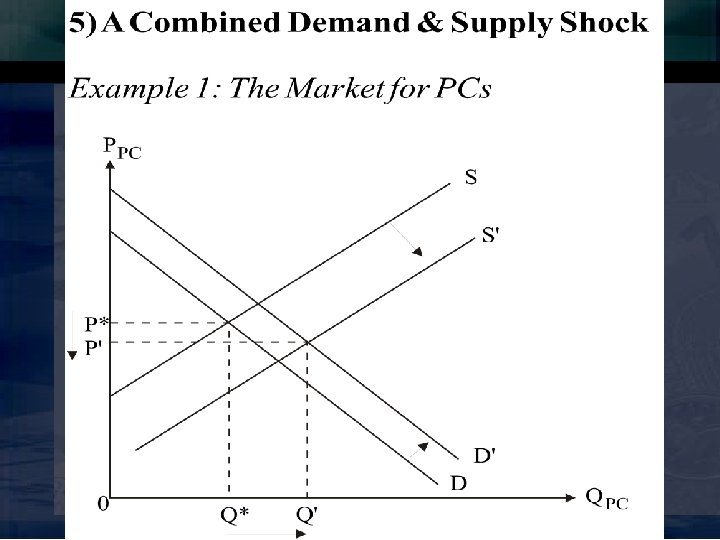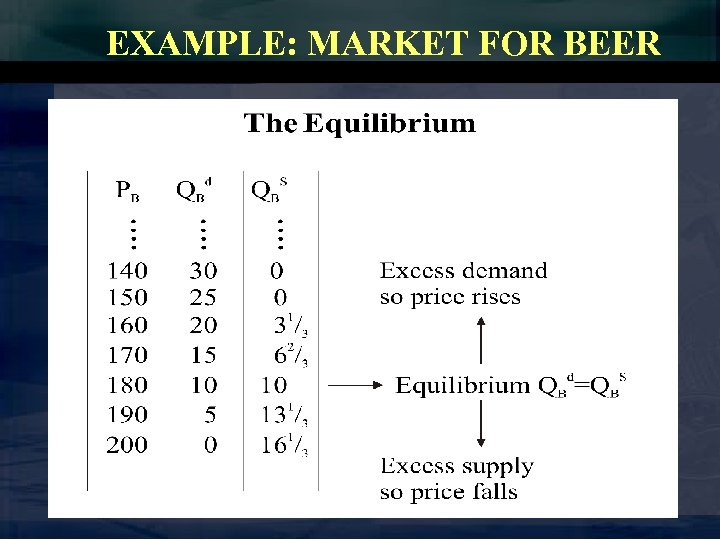EXAMPLE: MARKET FOR BEER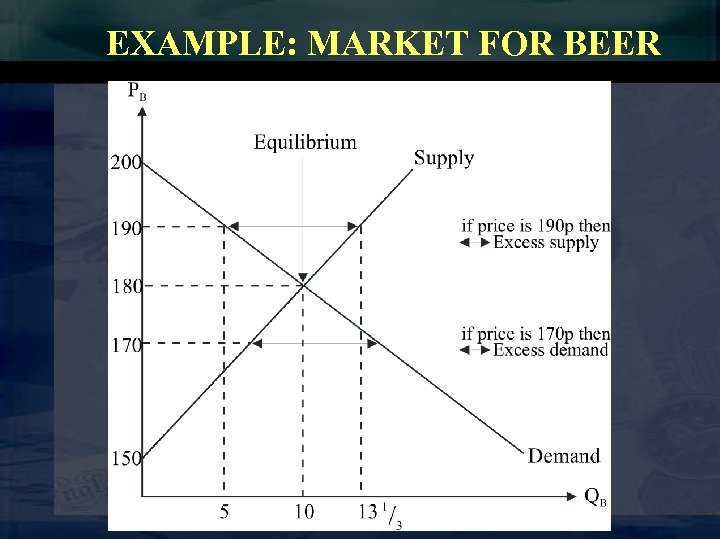EXAMPLE: MARKET FOR BEER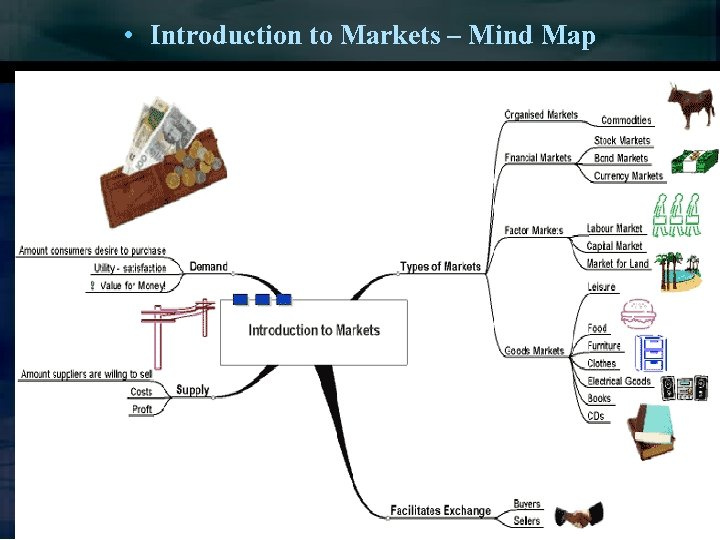• Introduction to Markets – Mind MapThe Market System – Mind Map